## Terms and Terminology Relating to Calculate Geometric Quantities

• Geometry: The branch of mathematics that addresses different shapes, angles, size and measurements.
• Straight angle: A straight angle is a flat line that is formed by two lines, or rays, at the common and shared vertex. A straight angle is 180 degrees.
• Right Angles: A right angle is an angle that is formed by two lines, or rays, at the common and shared vertex that is equal to 90 degrees.
• Acute angles: Acute angles are formed by two lines, or rays, at the common and shared vertex that are less than 90 degrees.
• Obtuse Angles: Angles that are formed by two lines, or rays, at the common and shared vertex that are more than and greater than 90 degrees but always less than 180 degrees which is a flat line.
• An adjacent angle: An angle that shares a common vertex side by side.
• Complementary angles: Two angles that, in combination with each other, add up to a total of 90 degrees.
• Supplementary angles: Two angles that, in combination with each other, add up to a total of 180 degrees.
• A rhombus: A polygon and a quadrilateral which is a shape that has four sides, and a shape with at least two parallel lines for its opposite sides, and having equal angles on, at least two, of its opposite sides.
• Perimeter: The measurement of the distance around a two dimensional shape. The circumference is the correlate for the perimeter of a circle.
• Circumference: The measurement of the distance around a circle. The perimeter is the correlate for circumference for quadrilaterals and triangles.
• Hypotenuse: The longest side of a triangle
• The Pythagorean Theorem: The length of one side squared + the length of the second side squared = The length of the hypotenuse squared
• Congruent triangles: A pair of triangles that have three sides that are of equal length when compared and contrasted to the three sides of the other congruent triangle AND that pair of triangles that also has three angles with the same number of degrees when compared and contrasted to the three angles of the other congruent triangle.
• Circle's center: The point that is precisely in the middle of the circle from which lines of equal length can be drawn to one side (radius) or extended to both sides of the circle (diameter).
• Diameter: The length of a straight line that passes through the center of the circle and extends in both directions to each side of the circle.
• Radius: The length of a straight line that begins at the center of the circle and extends in one direction to any one side of the circle. The radius of a circle is 1/2 of the length of the same circle's diameter.
• Segment: Any part or section of a circle that is outlined with one straight line that extends to two sides of the circle and an arc.
• Sector: A portion or part of a circle that is outlined by two radii (pleural of radius) that begin in the center of the circle and an arc, as shown in the picture above.
• Chord: A straight line that touches two points on the circle. This line does not have to pass through the center of the circle, like the diameter does.
• Arc: An arc is any arc like continuous line that is part of a circle and its outer border, as shown in the picture above.
• Mathematical constant: A number or value that never changes and one that does not vary despite the changing of a circle's size, for example.
• Pi: A mathematical constant that always remains the same. It is an irrational number that goes to infinity and is equal to 3.14159.
• Radical: The symbol for square root.
• The square of a number: The product when the number is multiplied by itself.
• Square root: The reverse of squaring a number.
• An acute trapezoid: A trapezoid that has two angles along its longer base that are two acute angles.
• An obtuse trapezoid: An obtuse trapezoid has two angles that are greater than 90 degrees.
• A right trapezoid: A trapezoid that has two right angles adjacent or next to each other. A right trapezoid is also referred to as a right angled trapezoid.
• An isosceles trapezoid: A special type of an acute trapezoid that has two of its opposite bases parallel and the two legs or sides of the trapezoid equal in terms of their length. See the picture below.
• A square: A quadrilateral with four equal sides and four equal angles.
• Quadrilateral: A shape that has four sides; a polygon with four sides
• Regular quadrilateral: A shape that has four sides that are all equal in terms of length and all equal angles
• Polygon: A shape with multiple sides
• Tetragon: A four sided polygon.
• Cartesian coordinate system: Standardized terminology for the identification of coordinates, which are the points where a data point on the x axis and the data point on the y axis are identical. These coordinates are identified and labeled with 2 numbers separated by a comma.
• Quadrant I: One of the four quadrants in the graph that has positive numbers along the horizontal x axis and positive numbers along the vertical y axis. Quadrant I is the upper right hand corner of a graph with four quadrants.
• Quadrant II: One of the four quadrants in the graph that has negative numbers along the horizontal x axis and positive numbers along the vertical y axis. Quadrant II is the upper left hand corner of a graph with four quadrants.
• Quadrant III: One of the four quadrants in the graph that has negative numbers along the horizontal x axis and negative numbers along the vertical y axis. Quadrant III is the lower left hand corner of a graph with four quadrants.
• Quadrant IV: One of the four quadrants in the graph that has positive numbers along the horizontal x axis and negative numbers along the vertical y axis. Quadrant IV is the lower right hand corner of a graph with four quadrants.

## The Basic Concepts of GeometryGeometry is a branch of mathematics that addresses different shapes, angles, size and measurements.

Some of the basic concepts of geometry include different shapes, different angles and different lines and different arcs.

## Shapes

Some of the shapes that you will review and learn about in this section of the TEAS examination review include:

Some of these shapes are somewhat similar to each other and others are quite different from each other. For example, a square shares similarities with a rectangle and a parallelogram, but circles are quite different from squares, rectangles and a parallelograms.

Below, we will outline and summarize some of the characteristics, similarities and differences among the various shapes listed above.

### Squares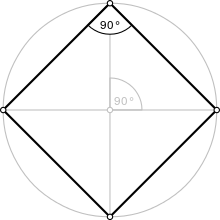A square is a special kind of rhombus. A rhombus is a polygon and a quadrilateral which is a shape that has four sides, and a shape with at least two parallel lines for its opposite sides, and having equal angles on, at least two, of its opposite sides. Other examples of shapes that are a rhombus are a:

• Trapezoid
• Parallelogram
• Rectangle

A polygon is a shape with multiple sides. A tetragon is a four sided polygon. A quadrilateral is a polygon with four sides, and a square is a polygon with four sides and four angles, but unlike a rectangle, a square is a regular quadrilateral, polygon that has four equal sides. A rectangle does not have four equal sides; instead a rectangle has four sides but two of these four sides are equal to each other in terms of length and the remaining two sides of the rectangle are equal to each other..

A square, simply stated, is a regular quadrilateral. A quadrilateral is a polygon shape that has four sides. A regular quadrilateral is a shape that has four sides that are all equal in terms of length and all equal angles. It is also referred to as a polygon because it is a shape with multiple sides.

Each of the angles in a square is 90 degrees. A 90 degree is referred to as a right angle, so a square has four right angles that are equal to each other. Additionally, a square has all sides perpendicular to its adjacent sides.

The diagonals of a square bisect, or split its right angles in half, and they also intersect and meet in the middle of the square at 90 degrees. The diagonals of a square is an X diagonally across it from the upper right hand corner of the square to the lower left hand corner of the square and from the upper left hand corner of the square to the lower right hand corner of the square, as shown below.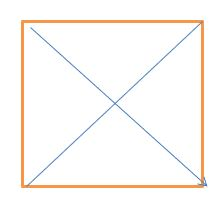In the picture above, the diagonals that bisect the square are shown in blue and the square itself is shown in orange.

The diagonals above divide, or bisect, the right angles in each of the square's corners into two equal angles, each of which are now 45 degrees and no longer 90 degrees. When these angles are equally bisected into two equal angles, each of these angles become 45 degrees or one half of 90 degrees.

Additionally, the four diagonals above, and in all squares are equal to each other in terms of their length and in terms of their angles.

You can also see that where the two diagonals cross each other in the middle of the square, there are four 90 degree angles.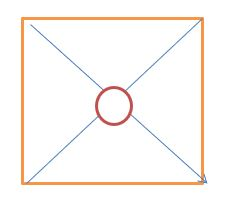In the picture above, the diagonals that bisect the square and the four 90 degree angles that are formed by the diagonal lines are covered with a red circle. Circles are 360 degrees and this circle represents the total degrees of the four angles, each of which are 90 degrees.

x degrees in a circle = 4 angles of 90 degrees each

x = 4 x 90

x = 360 degrees

#### Performing Geometric Calculations with Squares: Calculating the Perimeter of Squares

Squares are relatively simpler to perform calculations with when compared to other shapes because they have four equal sides.

Some of the calculations that you will be asked to do on your TEAS examination include:

• Calculating the perimeter of a square
• Calculating the area of a square
##### Calculating the Perimeter of a Square

The perimeter is defined as the area around a two dimensional shape. With the exception of a circle and circular shapes, whose perimeter is defined as its circumference. Everyday examples of when you will want to determine and calculate a perimeter or circumference include:

• The number of linear feet of fencing that you will need to put a fence around your backyard (perimeter)
• The number of linear feet of fencing that you will need to make a circular coral for your horses (circumference)
• The number of linear inches of fabric that you will need to place a decorative border around a rectangular tablecloth (perimeter)
• The number of linear inches of fabric that you will need to place a decorative border around a circular tablecloth (circumference)
• The number of linear feet of floor molding that you will need to trim around the dining room floor (perimeter)
• The number of linear feet of corner ceiling molding that you will need to trim around the ceiling of you bed room (perimeter)

Calculating the perimeter of a square can be done in several ways, but all calculations should be the same and identical in terms of the perimeter. The answers to these calculations can be done by:

• Adding the length of the four sides of the square together
• Multiplying the length of one side of the square by 4 because each of the four sides of a square are equal to each other. This multiplication by 4 cannot be done with rectangles or any other shape unless it is a square because, by the very nature of a square, all the four sides of a square are equal to each other.

Here is an example of calculating the perimeter of a square:If each side of the square above is 8 inches, the perimeter of the square is:

• x inches = 8 + 8 + 8 + 8
• x inches = 32 inches
• Answer - The perimeter of the square is 32 inches or 32 linear inches

----------

Because each of the four sides of a square are equal and exactly the same, the perimeter of this same square with each side as 8 inches can be calculated also as below:

• x inches = 8 x 4
• x = 32 inches
• Answer - The perimeter of the square is 32 inches or 32 linear inches

----------

If each side of the square above is 6 inches, the perimeter of the square is:

• x = 6 inches x 4
• x = 24 inches
• Answer - The perimeter of the square is 24 inches or 24 linear inches

----------

Again, because each of the four sides of a square are equal and exactly the same, the perimeter of this same square with each side as 6 inches can be calculated also as below:

• x inches = 6 x 4
• x = 24 inches
• Answer - The perimeter of the square is 24 inches or 24 linear inches

----------

##### Performing Geometric Calculations with Squares: Calculating the Area of Squares

Calculating the area of a square is quite dissimilar to the calculation of the perimeter of a square. The area of a square and other shapes is not the border around the shape, instead, the area of a square is the space inside of all the exterior borders or perimeter.

For example, the shape below is a square with an orange border or perimeter.The shape below is the same square but the interior of the square is colored in blue. This blue colored section below is the area of the square.Similarly, the shape below is a triangle with an orange border or perimeter.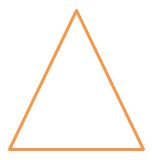The shape below is the same triangle but the interior of the triangle is colored in blue. This blue colored section below is the area of the triangle.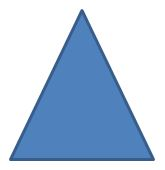Everyday examples of when you will want to determine and calculate the area of a shape include:

• The number of square feet of grass seed that you will need to seed your backyard
• The number of square feet of concrete that you will need to make a patio in your yard
• The number of square inches of fabric that you will need to make a tablecloth
• The number of square feet of flooring that you will need to buy for a new dining room floor
• The number of square feet of tile that you will need to buy in order to lay a new floor in the family room
• The number of square feet of sheet rock or drywall you will have to purchase in order to replace your water damaged ceiling in the living room

Calculating the area of a square can be done in several ways, but all calculation methods should yield the same and identical calculation answer in terms of the area. Additionally, unlike the perimeter which measures things in units of measurement like inches, feet and yards, linear inches, linear feet and linear yards, the answer to area calculations are expressed as square inches, square feet and square yards for all shapes including a square, rectangle and triangle

The method for calculating the area of a square is multiplying the length of the square by the width or height of the square OR by simply multiplying the length or width by itself. Here is an example of finding the area of a square. The area of a square, therefore, is the product of the length and width of the square.

The area of a square that is 5 foot by 5 foot which is a square that is 5 foot x 5 foot x 5 foot x 5 because a square has four equal sides is calculated as: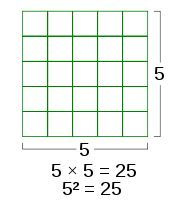5 feet x 5 feet = 5 square feet

Here are some other examples of calculating the area of different squares.

• The area of a 12 inch square:
• 12 x 12 = 144
• Answer - 144 square inches

----------

• The area of a 4 inch square:
• 4 x 4 = 16
• Answer - 16 square inches

----------

• The area of a 9 inch square:
• 9 x 9 = 81
• Answer - 81 square inches

----------

• The area of a 2 foot square:
• 2 x 2 = 4
• Answer - 4 square feet

----------

• The area of a 11 meter square:
• 11 x 11 = 121
• Answer - 121 square meters

----------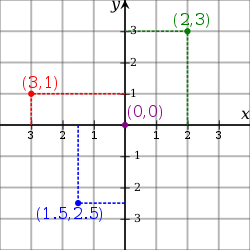Illustration of a Cartesian coordinate plane. Four points are marked and labeled with their coordinates: (2, 3) in green, (−3, 1) in red, (−1.5, −2.5) in blue, and the origin (0, 0) in purple.

In the section immediately above, you should have learned and mastered the concepts underlying the calculations used to determine the area of a square. Your TEAS examination may have variations of these basic underlying concepts with things like word problems and the determination of areas using the Cartesian coordinate system, as fully described in the section entitled "Measurement and Data: M 2; Objective 1: Interpreting Relevant Information From Tables, Charts and Graphs.

Standardized terminology for the identification of coordinates, which are the points where a data point on the x axis and the data point on the y axis are identical. These coordinates are identified and labeled with 2 numbers separated by a comma. One of these numbers is the point where the horizontal line, or x axis, is from the point of origin is and the other number the point where the vertical line from the point of origin is. It is shown as x, y.

The point of origin is 0, 0

This Cartesian coordinate system is used to interpret data on a graph and also to construct a graph and a shape, such as a square and a rectangle, by plotting points on the graph.

Examples of coordinates are:

• 4,3 where 4 is on the x axis and 3 is on the y axis
• 5,9 where 5 is on the x axis and 9 is on the y axis
• 6, 2 where 6 is on the x axis and 2 is on the y axis
• 0,0 where 0 is on the x axis and 0 is on the y axis and this is the point of origin on a four quadrant graph
• -3, 8 where - 3 is on the x axis and 8 is on the y axis
• 8, -3 where 8 is on the x axis and -3 is on the y axis
• -3, -5 where -3 is on the x axis and -5 is on the y axis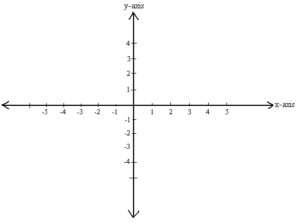Looking at the graph and the coordinates in the picture above you can calculate the area of a square as based on the Cartesian coordinates.

For example, the Cartesian coordinates 3, 3 are in Quadrant I so, draw a square with these coordinates in Quadrant I. Each side of this square is precisely 3, so the perimeter of this square is:

3 + 3 + 3 + 3 = 12

OR

3 x 4 = 12

And the area of this square with the coordinates of 3, 3 is calculated as:

3 x 3 = 9

Here are some more examples of determining the perimeters and areas of squares with the following coordinates:

• (4, 4)

Perimeter of the Square:

• x = 4 + 4 + 4 + 4
• x = 16

Area of the Square:

• 4 x 4 = 16

----------

• (7, 7)

Perimeter of the Square:

• x = 7 + 7 + 7 + 7
• x = 28

Area of the Square:

• 7 x 7 = 49

----------

### Rectangles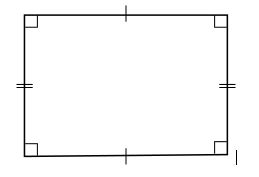A rectangle is identical to a square in terms of some of its characteristics and unlike a square in terms of other characteristics.

The similarities between squares and rectangles include the facts that both squares and rectangles are:

• Four sided shapes
• A special kind of rhombus
• Polygons
• Tetragons
• Made of four right angles, each of which are 90 degrees
• Have opposite sides that are equal to each other in terms of their length
• Have adjacent sides that are perpendicular to each other
• Able to be bisected, or split in half at its right angles with a diagonal
• When bisected, or split in half at its right angles in each of the four corners with a diagonal, each of these newly formed 8 angles will be 45 degrees which will still total 90 degree at each of the four bisected corners
• When bisected, interior angles where these diagonals intersect and meet in the middle of the square or rectangle
• The interior angles that are formed with the diagonals will total 360 degrees

The differences between squares and rectangles include the facts that:

• Squares have four equal sides and rectangles do not.
• Rectangles have pairs of equal sides but NOT four equal sides.
• Squares are a regular quadrilateral meaning that all sides are equal to each other.
• Rectangles are not regular quadrilaterals because all sides are not equal to each other
• Although both squares and rectangles have interior angles that total 360 degrees when bisected with diagonal lines, squares form four 90 degree angles in the interior and rectangles form four angles other than 90 degree angles in the interior

Rectangles and their interior angles, when bisected with diagonals, will be discussed below.

Rectangle 1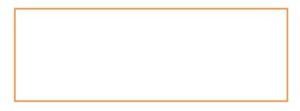Rectangle 2Rectangle 3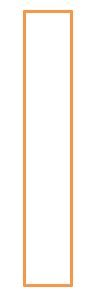As you can see in the three pictures above, these 3 rectangles look quite different from each other, however, they are all rectangles are quite different in terms of their lengths and widths as well as their forms and shapes.

They are all rectangles, because, as you should be able to see in the illustrations of the rectangles above, all of these rectangles:

• Have four sides
• Have four right angles, each of which is 90 degrees, in each of its four corners
• Have opposite sides that are equal to each other in terms of their length but not 4 equal sides
• Able to be bisected, or split in half at its right angles with a diagonal
• When bisected, or split in half at its right angles with a diagonal, have a total of 360 degree interior angles where these diagonals intersect and meet in the middle of the rectangle, but unlike squares, these angles are NOT all 90 degrees.

When comparing Rectangle 2 to Rectangles 1 and 3, you may, at first glance, think that this rectangle is a square instead of a rectangle but it is not. It is close to a square but it is not a square because a square must have 4 sides that are precisely equal and the sides on the Rectangle 2 are not precisely equal. At times, it is necessary to measure the sides of rectangles and squares to determine if the shape is a square or a rectangle.

If all four of the sides are precisely equal when you measure them, the shape is a square. On the other hand, if all four sides are not precisely equal when you measure them, the shape is not a square, it is something other than a square and it may be a rectangle. To then determine if this shape, which was determined to be not a square is indeed a rectangle, you will have to carefully measure its opposite sides. If the opposite sides of the shape are precisely equal when you measure them, the shape is a rectangle but if each pair of opposite sides is not precisely equal when you measure them, it is not a rectangle.

These measurements are typically not indicated when a shape such as Rectangles 1 and 3 are obviously the same in terms of the lengths of their opposite sides of their length and width, but there still may be times that the measurements of the angles for what appears to be a rectangle or square is not so because their 4 angles are not precisely 90 degrees.

A protractor, such as the ones shown in the picture below, is used to measure angles and the hand held tool that is used to confirm whether or not all angles of a rectangle are 90 degrees.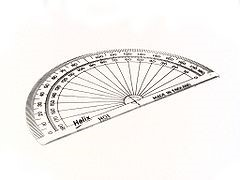A half circle protractor marked in degrees (180°).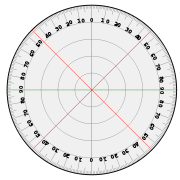A 360° protractor marked in degrees.

#### The Diagonals of a Rectangle

Rectangle 1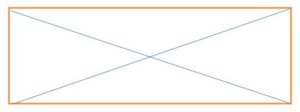Rectangle 2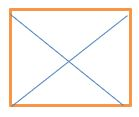Rectangle 3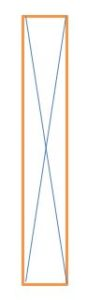As you can see above for Rectangle 1, Rectangle 2 and Rectangle 3, the diagonals of these rectangles are equal in terms of their length and they bisect the corner right angles into two equal angles of 45 degrees each in every one of the four corners of these rectangles.

Rectangle 1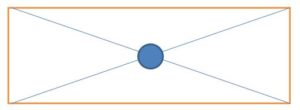Rectangle 2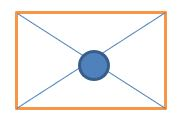Rectangle 3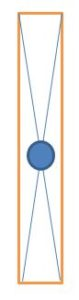Lastly, as you can see above for Rectangle 1, Rectangle 2 and Rectangle 3, the diagonals of these rectangles that bisect the corner right angles insect and cross each other forming a 360 degree angle just as occurs with squares, however, unlike a square these interior angles are not all 90 degree or right angles.

In Rectangle 1, you can see that the top and bottom angles of this bisected rectangle are equal and you should also be able to see that the right and left side angles of this bisected rectangle are equal and that all of these 4 interior angles combine into a circle of 360 degrees.

You should also be able to see that the top and bottom angles of this bisected rectangle are obtuse angles greater than 90 degrees and you should also be able to see that the right and left side angles of this bisected rectangle are acute angles of less than 90 degrees.

In Rectangle 2, you can see that the top and bottom angles of this bisected rectangle are equal and you should also be able to see that the right and left side angles of this bisected rectangle are equal and that all of these 4 interior angles combine into a circle of 360 degrees. Although more difficult to see and determine without measurements, the top and bottom angles of this bisected rectangle are obtuse angles slightly greater than 90 degrees and you should also be able to measure and see that the right and left side angles of this bisected rectangle are acute angles of slightly less than 90 degrees.

Far more obvious than Rectangle 2, in Rectangle 3, you can see that the top and bottom angles of this bisected rectangle are equal and you should also be able to see that the right and left side angles of this bisected rectangle are equal and that all of these 4 interior angles combine into a circle of 360 degrees.

You should also be able to see that the top and bottom angles of this bisected rectangle are very acute angles less than 90 degrees and you should also be able to see that the right and left side angles of this bisected rectangle are very obtuse angles of more than 90 degrees.

##### Performing Geometric Calculations with Rectangles: Calculating the Perimeter of Rectangles

Rectangles, like squares are relatively simpler to perform calculations with when compared to other shapes because they have four equal sides.

Some of the calculations that you will be asked to do on your TEAS examination include:

• Calculating the perimeter of a rectangle
• Calculating the area of a rectangle
##### Calculating the Perimeter of a Rectangle

Again, the perimeter is defined as the area around a two dimensional shape.

Everyday examples of when you will want to determine and calculate a perimeter of a rectangle include the determination of:

• The number of linear feet of fencing that you will need to put a fence around your backyard (perimeter)
• The number of linear inches of fabric that you will need to place a decorative border around a rectangular tablecloth (perimeter)
• The number of linear feet of floor molding that you will need to trim around the dining room floor (perimeter)
• The number of linear feet of corner ceiling molding that you will need to trim around the ceiling of you bed room (perimeter)

Calculating the perimeter of a rectangle can be done in several ways, but all calculations should be the same and identical in terms of the perimeter. The answers to these calculations can be done by:

• Adding the length of the four sides of the square together
• Multiplying the length of one side of the square by 4 because each of the four sides of a square are equal to each other. This multiplication by 4 cannot be done with rectangles or any other shape unless it is a square because, by the very nature of a square, all the four sides of a square are equal to each other.Here are some examples of calculating the perimeter of a rectangle:

Example 1

If the length of the rectangle above is 8 inches and the width of the rectangle above is 6 inches, the perimeter of this rectangle is:

• x inches = 8 + 8 + 6 + 6
• x inches = 16 + 12
• x inches = 28 inches

OR

• x inches = 8 x 2 + 6 x 2
• x = 16 + 12
• x inches = 28 inches

Answer - The perimeter of this rectangle is 28 inches or 28 linear inches

----------

Example 2

If the length of the rectangle above is 7 inches and the width of the rectangle above is 4 inches, the perimeter of this rectangle is:

• x inches = 7 + 7 + 4 + 4
• x inches = 14 + 8
• x inches = 22 inches

OR

• x inches = 7 x 2 + 4 x 2
• x = 14 + 8 = 22 inches

Answer - The perimeter of this rectangle is 22 inches or 22 linear inches

----------

Example 3

If the length of the rectangle above is 2 inches and the width of the rectangle above is 1 inch, the perimeter of this rectangle is:

• x inches = 2 + 2 + 1 + 1
• x inches = 4 + 2
• x inches = 6 inches

OR

• x inches = 2 x 2 + 2 x 1
• x = 4 + 2
• x = 6 inches

Answer - The perimeter of this rectangle is 6 inches or 6 linear inches

----------

Calculating a part or portion of the perimeter of a rectangle is a little more complicated and complex than simply calculating the perimeter of the entire rectangle, however, it is still relatively simple.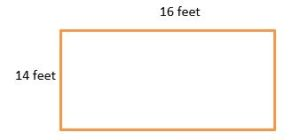If you have a rectangle, for example, that is 16 feet in length and 12 feet in height, and you are asked to determine the perimeter of only ½ of this rectangle , as shown in the picture above, you can perform this calculation as below.

Because a rectangle has equal opposite sides, you could simply divide the lengths of 2 sides by 2 and then divide the two heights by 2 to calculate the perimeter of ½ of the rectangle.

The perimeter of ½ of this rectangle = 16 ÷ 2 + 16 ÷ 2 + 12 ÷ 2 + 12 ÷ 2

• 2 x feet = 8 + 8 + 6 + 6
• 2 x feet = 16 + 12
• 2 x = 28 feet
• x feet = 28 ÷ 2
• x feet = 14 feet
• Answer - 14 feet or 14 linear feet
##### Performing Geometric Calculations with Rectangles: Calculating the Area of Rectangles

Calculating the area of a rectangle is quite dissimilar to the calculation of the perimeter of a rectangle. The area of a rectangle and other shapes is not the border around the shape, instead, the area of a rectangle is the space inside of all the exterior borders or perimeter.

For example, the shape below is a rectangle with an orange border or perimeter around it.The shape below is the same rectangle but the interior of the rectangle is colored in blue. This blue colored section below is the area of the rectangle.Everyday examples of when you will want to determine and calculate the area of a rectangle and other shapes include:

• The number of square feet of grass seed that you will need to seed your backyard
• The number of square feet of concrete that you will need to make a patio in your yard
• The number of square inches of fabric that you will need to make a tablecloth
• The number of square feet of flooring that you will need to buy for a new dining room floor
• The number of square feet of tile that you will need to buy in order to lay a new floor in the family room
• The number of square feet of sheet rock or drywall you will have to purchase in order to replace your water damaged ceiling in the living room

Calculating the area of a rectangle can be done in several ways, but all calculation methods should yield the same and identical calculation answer in terms of the area. Additionally, unlike perimeter which measures in units of measurement like inches, feet and yards, linear inches, linear feet and linear yards, the answer to area calculations are expressed as square inches, square feet and square yards for all shapes including a rectangle, a square and other shapes.

The method for calculating the area of a rectangle is multiplying the length of the rectangle by the width or height of the rectangle. The area of a rectangle, therefore, is the product of the length and width of the rectangle which is exactly identical to the procedure that is done to calculate and determine the area of a square, as fully discussed above.

Here are some other examples of calculating the area of different rectangles.

• The area of a rectangle that is 10 by 8 inches:
• 10 x 8 = 80
• Answer - 80 square inches

----------

• The area of a 4 by 22 inch rectangle:
• 4 x 22 = 88
• Answer - 88 square inches

----------

• The area of a 12 by 11 inch rectangle:
• 12 x 11 = 132
• Answer - 132 square inches

----------

• The area of a rectangle that measures 12 feet by 8 feet:
• 12 x 8 = 92
• Answer - 96 square feet

----------

###In the section immediately above, you should have learned and mastered the concepts underlying the calculations used to determine the area of a rectangle. Your TEAS examination may have variations of these basic underlying concepts with things like word problems and the determination of areas using the Cartesian coordinate system, as fully described in the section entitled "Measurement and Data: M 2; Objective 1: Interpreting Relevant Information From Tables, Charts and Graphs". These TEAS examination expectations are similar in terms of squares, as discussed in the section immediately above this.Looking at the graph and the coordinates in the picture above you can calculate the area of a rectangle as based on the Cartesian coordinates and in the same manner that these calculations are done with squares, as discussed in the section above.

For example, the Cartesian coordinates 3, 4 are in Quadrant I so, draw a rectangle with these coordinates in Quadrant I. Two sides of this rectangle are precisely 3 and the other two sides of this rectangle are precisely 4, so the perimeter of this rectangle is:

3 + 3 + 4 + 4 = 14

And, the area of this rectangle with the coordinates of 3, 4 is calculated as:

3 x 4 = 12

Here are some more examples of determining the area and perimeter of rectangles with the following coordinates:

Example 1

• (4, 6)

Perimeter of the Rectangle:

• x = 4 + 4 + 6 + 6
• x = 20

Area of the Rectangle:

• 4 x 6 = 24

-----------

Example 2

• (6, 8)

Perimeter of the Rectangle:

• x = 6 + 6 + 8 + 8
• x = 28

Area of the Rectangle:

• 6 x 8 = 48

-----------

Example 3

• (8, 10)

Perimeter of the Rectangle:

• x = 8 + 8 + 10 + 10
• x = 36

Area of the Rectangle:

• 8 x 10 = 80

-----------

### Triangles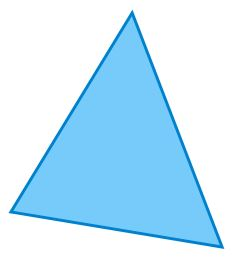A triangle is a polygon with three edges and three vertices. It is one of the basic shapes in geometry. A triangle with vertices A, B, and C is denoted

Simply stated, a triangle is a polygon with three sides and three internal vertices or angles that add up to 180 degrees.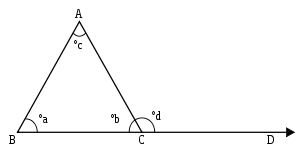Triangles also have exterior angles as shown in the picture above. Because the total of the internal angles of a triangle total 180 degrees, these external angles, as shown in the diagram above, total 360 degrees.

The several types and classifications of triangles are the:

• Equilateral triangle
• Isosceles triangle
• Scalene triangle
• Right triangle
• Isosceles right triangle
• The 30 – 60 – 90 triangle
• Oblique triangle
• Acute triangle
• Obtuse triangle

#### Equilateral Triangles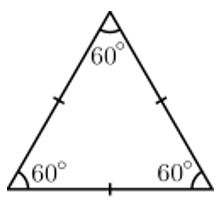Equilateral triangles, as shown in the picture above, are characterized with:

• Equal sides in terms of their length
• Three angles all of which are 60 degrees

Not only are equilateral triangles polygons like all other triangles, equilateral triangles are also referred to as a regular polygon, like a square and a rectangle are, because all of their angles are equal.

#### Isosceles Triangles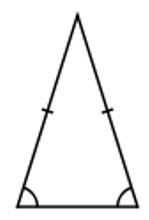Isosceles triangles, are similar to equilateral triangles in some respects, but they are also different from equilateral triangles in other respects.

Both equilateral triangles and isosceles triangles are polygons with 3 sides and 3 angles and both of these types of triangles have a total of 180 degrees; however, these two types of triangles are also different.

Isosceles triangles:

• Have two of their three angles as equal and the equilateral triangle has all three equal angles.
• Have two of their three sides as equal and the equilateral triangle has all three equal sides.

In the picture of the isosceles triangle above, you can see that the two sides of the triangle are equal in terms of their length and NOT the same as the base of the above triangle. You will also see that the two angles at the base of the above isosceles triangle are equal, but the top angle of this triangle is not the same as the two angles at the base of the above isosceles triangle.

A special isosceles triangles is a 45 – 45 – 90 degree triangle that has two equal angles of 45 degrees and one angle of 90 degrees with a total of 180 degrees, as all triangles have.

#### Scalene Triangles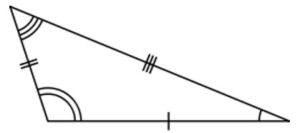Scalene triangles are the least organized of all the different types of triangles. Although scalene triangles are three sided polygons with 3 angles and a total of 180 degrees, scalene triangles have three different lengths for their 3 sides and they also have three different angles. NONE of its sides are equal in length and NONE of its angles are equal.

The picture above clearly shows the three different lengths for its 3 sides and the three different angles.

#### Right Triangles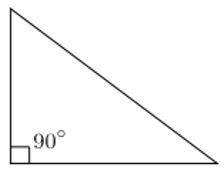Right triangles have one 90 degree right angle and 2 other interior acute angles that are complementary, which, when added to the 90 degree right angle, will total 180 degrees. As you may recall, complementary angles are two angles that, when combined and added together, total 90 degrees. Based on these facts that a right triangle has one 90 degree angle and the other two angles are complementary and add up to 90 degrees, the total internal angles for a right triangle is 180 degrees as all triangles are.

The long line of the right triangle opposite the 90 degree right angle of this triangle is the longest line of this triangle and this line is called the hypotenuse of the triangle.

##### The Pythagorean Theorem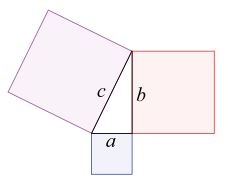These triangles are special 3-4-5 triangles that follow the Pythagorean Theorem

The Pythagorean Theorem states that:

The length of one side squared + the length of the second side squared = The length of the hypotenuse squared

OR

A squared + B squared = C squared, as show in the colored diagram above.

OR

c 2 = a2 + b2 where c is the length of hypotenuse and a and b are the lengths of the two other sides of the right triangle, as shown in the colored diagram above.

Here are some examples of applying the Pythagorean Theorem to right triangles.

Example 1:

One side of a right triangle measures 6 feet and the hypotenuse measures 10 feet. What is the length of the remaining side of this right triangle?

To solve this problem, you would use the Pythagorean Theorem rule of c 2 = a2 + b2 where c is the length of hypotenuse and a and b are the lengths of the two other sides of the right triangle.

• c 2 = a 2 + b 2
• 10 2 = 62 + x 2
• 100 = 36 + x2
• x2 = 100 - 36
• x2 = 64
• x = 8 because the square root of 64 is 8 (8 x 8 = 64)
• Answer - The length of the remaining side of a right triangle is 8 feet when one side of the right triangle measures 6 feet and the hypotenuse measures 10 feet.

Example 2:

One side of a right triangle measures 12 inches and the other side measures 9 inches. What is the length of the hypotenuse of this right triangle?

To solve this problem, you would use the Pythagorean Theorem rule of c 2 = a2 + b2 where c is the length of hypotenuse and a and b are the lengths of the two other sides of the right triangle.

• c 2 = a 2 + b 2
• c 2 = 122 + 92
• c 2 = 144 + 81
• c 2 = 225
• c 2 = 15 because the square root of 225 is 15 (15 x 15 = 225)

#### Isosceles Right Triangle

An isosceles right triangle is a combination of and variation of the standard isosceles triangle and the right triangle. An isosceles right triangle is a triangle has a 90 degree angle, which is a characteristic of the right triangle, and it also has sides and angles that are proportionate.

#### The 30 – 60 – 90 Triangle

The 30 – 60 – 90 triangle is a variation of the standard right triangle. A 30 – 60 – 90 triangle has one right angle of 90 degrees and the two other angles are 30 degrees and 60 degrees.

#### Oblique Triangles

An oblique triangle is a triangle, like all other triangles, that is a polygon with three sides and three vertices or angles that add up to 180 degrees.

What makes oblique triangles distinctly different from and dissimilar to other triangles is the fact that oblique triangles do NOT have one angle that is 90 degrees.

#### Acute Triangles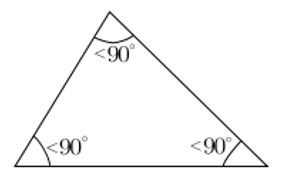Acute triangles, like the one shown above, are triangles that do NOT have at least one 90 degree interior angle. Although the total number of degrees for an acute triangle add up to 180 degrees, none of these interior angles is 90 degrees,

#### Obtuse Triangles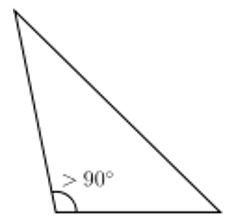Obtuse triangles are triangles that have one interior angle that is greater than 90 degrees, as shown in the diagram above.

##### Congruent and Similar Triangles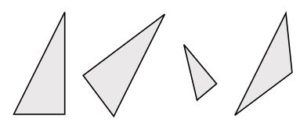An example of congruence. The two triangles on the left are congruent, while the third is similar to them. The last triangle is neither similar nor congruent to any of the others. Note that congruence permits alteration of some properties, such as location and orientation, but leaves others unchanged, like distance and angles. The unchanged properties are called invariants.

Triangles can be described as congruent and similar. The determination of congruence is more rigorous than the determination of similarity according to established criteria.

Quite often, the term equal and the term congruent are used interchangeably; however, they are slightly different.

As shown in the picture above, the first two triangles are congruent despite the fact that, in contrast to the first triangle, is rotated and turned in a different direction. What these first two triangles share in common is that their lines, or line segments, are the same length and their angles are the same in terms of their degrees.

Other shapes, like a circle, which do not have possible variations in terms of their total degrees because all true circles are 360 degrees and no more or no less can be also congruent but not according to the criteria for congruency stated above for triangles. Instead, for example, circles are deemed congruent when they have the same diameter.

The diameter of a circle is defined as the length of a line that passes through the center of the circle to both interior sides of the circle, as shown in the picture below in cyan or a light blue-green color.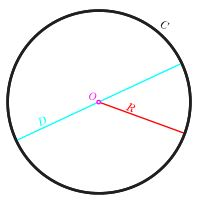Circle with circumference (C) in black, diameter (D) in cyan, radius (R) in red, and center or origin (O) in magenta.

Similarity, in contrast to congruency, applies to some shapes that are similar but not congruent because they lack the more rigorous and restrictive criteria for congruency. Similarity applies to triangles and other shapes that are, for example, the same in terms of their shape, but they are not congruent because they lack the same size.

The properties of a shape, such as a triangle, that cannot change or be different among triangles are referred to as invariants. For example, distance and angles cannot be different to deem these shapes as congruent, but on the other hand, things like size can differ among the shapes and congruency can still be present.In the above picture, the third triangle is NOT congruent with the first two triangles that are congruent with each other, because the third triangle is not the same size, or distance, although its angles appear to be the same as the angles on the first two congruent triangles.

In summary, triangles are congruent when they have precisely the same size and shape. This confirmation, as discussed above, should be measured with a protractor because it is often not possible to be certain of congruency without precise and accurate measurements of the three sides and the three angles of the triangle.

In essence, the criteria for congruent triangles include these 6 criteria:

1. Side 1 of each of the triangles must be identical and the same in terms of their length.
2. Side 2 of each of the triangles must be identical and the same in terms of their length.
3. Side 3 of each of the triangles must be identical and the same in terms of their length.
4. Angle 1 of each of the triangles must be identical and the same in terms of their degrees.
5. Angle 2 of each of the triangles must be identical and the same in terms of their degrees.
6. Angle 3 of each of the triangles must be identical and the same in terms of their degrees.
##### Performing Geometric Calculations with Triangles: Calculating the Perimeter of Triangles

Some of the calculations that you will be asked to do on your TEAS examination include:

• Calculating the perimeter of a triangle
• Calculating the area of a triangle

The concepts underlying the calculation of the perimeter of a triangle are the same as the concepts underlying the calculations of the perimeters of rectangle and squares. However, unlike calculating the perimeters of rectangles and squares, the calculation of the perimeter of a triangle has no short cuts like multiplying the length of one side of a square times 4 or multiplying the opposite sides of a rectangle times 2 and then adding these two products together.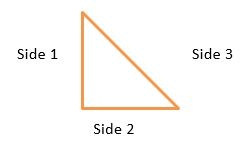The perimeter of a triangle entails the addition of the lengths of each of the three sides of the triangle, as shown in the diagram above where the orange lines that outline the triangle is the perimeter of the triangle.

For example:

The perimeter of a triangle that has three sides measuring 1 inch, 6 inches and 8 inches is done by adding 1 and 6 and 8 to get the sum of 15 inches.

Here are some more examples:

Example 1:

Calculate the perimeter of a triangle that has these measurements:

• Side 1 measures 6 cm
• Side 2 measures 8 cm
• Side 3 measures 13 cm
• x cm = 6 cm + 8 cm + 13 cm
• x cm = 27 cm
• Answer - The perimeter of this triangle is 27 cm or 27 linear cm.

----------

Example 2:

Calculate the perimeter of a triangle that has these measurements:

• Side 1 measures 5 feet
• Side 2 measures 9 feet
• Side 3 measures 2 feet
• x feet = 5 feet + 9 feet + 2 feet
• x feet = 16 feet
• Answer - The perimeter of this triangle is 16 feet or 16 linear feet

----------

Example 3:

Calculate the perimeter of a triangle that has these measurements:

• Side 1 measures 1 meter
• Side 2 measures 19 meters
• Side 3 measures 16 meters
• x meters = 1 meter + 19 meters + 16 meters
• x meters = 36
• Answer - The perimeter of this triangle is 36 meters or 36 linear meters

----------

Example 4:

Calculate the perimeter of a triangle that has these measurements:

• Side 1 measures 16 inches
• Side 2 measures 1.5 feet
• Side 3 measures 8 inches

This example entails more than simply adding like and identical measurement units like cm or meters. This example has the dissimilar measurement units of feet and inches. So, as you have learned previously in this TEAS review, you must convert all measurement units to the same measurement unit before you can accurately perform the calculation.

In this example, you must convert all the measurements into either inches or feet.

For the purpose of this example, we will convert and use only inches, although you could also convert and use only feet as well. Because side 1 and side 2 of the triangle are in inches, you will only have to convert the measurement of side 2 into inches as follows, using ratio and proportion, as was fully discussed in a previous section of this TEAS review.

• 12 inches : 1 foot = x inches : 1.5 feet
• x inches = 12 x 1.5
• x inches = 16 inches

There are 16 inches in 1.5 feet, so you can now calculate the perimeter of this triangle using inches as the only unit of measurement because the feet unit of measurement was converted to the inches unit of measurement.

• x inches = 16 inches + 16 inches + 8 inches
• x inches = 40
• Answer - The perimeter of this triangle is 40 inches or 40 linear inches

----------

Example 5:

Calculate the perimeter of a triangle that has these measurements:

• Side 1 measures 2 km
• Side 2 measures 2 miles
• Side 3 measures 5 km

Similar to the example immediately above, this example has two different measurement units. These measurement units are km (kilometer) and miles.

In this example, you must convert all the measurements into either km or miles before can accurately perform this calculation.

For the purpose of this example, we will convert and use only kilometers, although you could also convert and use only miles as well. Because side 1 and side 3 of the triangle are in kilometers, you will only have to convert the measurement of side 2 into km as follows, using ratio and proportion, as was fully discussed in a previous section of this TEAS review.

• 1.61 km : 1 mile = x km : 2 miles
• x km = 1.61 x 2
• x km = 3.22
• x = 3.22 km

There are 3.22 km in 2 miles, so you can now calculate the perimeter of this triangle using kms as the only unit of measurement because the miles unit of measurement was converted to the kilometers unit of measurement.

• x km = 2 km + 3.2 km + 5 km
• x km = 10.2 km
• Answer - The perimeter of this triangle is 10.2 km or 10.2 linear km

Everyday examples of when you will want to determine and calculate a perimeter of a triangle include the determination of:

• The number of linear feet of fencing that you will need to put a fence around your triangular shaped garden
• The number of linear inches of fabric that you will need to trim a holiday triangular tree shaped tree

Here are two examples of everyday problems that you will want to determine and calculate:

Example 1:

How many linear feet of fencing that you will need to put a fence around your triangular shaped garden when it measures as shown in the triangle below?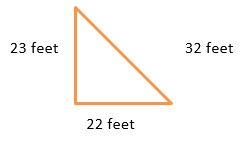• Perimeter = Side 1 + Side 2 + Side 3
• x feet = 23 feet + 22 feet + 32 feet
• x feet = 77 feet
• Answer - The perimeter of this triangle is 77 feet or 77 linear cm.

-----------

Example 2:

How many linear inches of fabric that you will need to trim a holiday triangular tree shaped tree like the one shown below?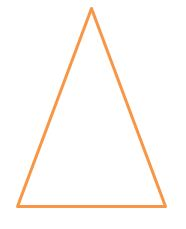• Perimeter = Side 1 + Side 2 + Side 3
• x inches = 24 inches + 24 inches + 16 inches
• x inches = 64 inches
• Answer - The perimeter of this triangle is 64 inches or 64 linear inches.
##### Performing Geometric Calculations with Triangles: Calculating the Area of Triangles

Calculating the area of a triangle is unlike the calculations relating to the areas of a rectangle and a square. As you should recall, the areas of a rectangle and a square are calculated by multiplying the length and width, or height, of the rectangle or square.

Calculating the area of a triangle is done using the mathematical or geometrical formula or rule of:

Area of a triangle 1/2 length of the base of the triangle x the length of the height of the triangle.

OR

Area = 1/2 bh where b is the length of the base and h is the length of the height of the triangle

Example 1:

Find the area of the triangle below.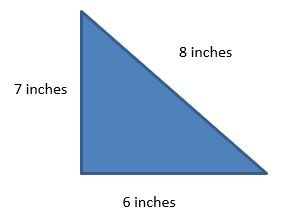• Area 1/2 bh where b is the length of the base and h is the length of the height of the triangle

OR

• Area = bh/2
• Area = 6 x 7/2
• Area = 42/2
• Area = 21 square inches
• Answer - The area of this triangle is 21 square inches.

----------

Example 2:

Find the area of the triangle below.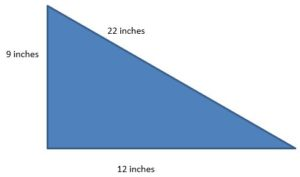• Area = bh/2
• Area = 12 x 9/2
• Area = 108/2
• Area = 54 square inches
• Answer - The area of this triangle is 54 square inches.

----------

Example 3:

Find the area of the triangle below.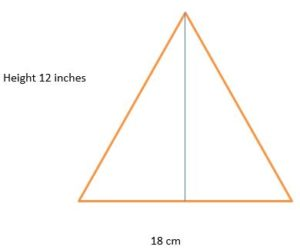This triangle has a blue line that is perpendicular to the base of the triangle which indicates the height of this triangle as 12 inches.

This example entails more than simply multiplying like and identical measurement units like cm or meters. This example has the dissimilar measurement units of cm and inches. So, as you have learned previously in this TEAS review, you must convert all measurement units to the same measurement unit before you can accurately perform the calculation.

For the purpose of this example, we will convert and use only inches, although you could also convert and use only cm as well unless the TEAS examination question asks for you to use a specific unit of measurement.

Using ratio and proportion, as was fully discussed in a previous section of this TEAS review, you would perform this calculation as below.

• 2.54 cm : 1 inch = x cm : 12 inches
• x = 2.54 x 12
• x = 30.48 cm
• The height of this triangle is 30.48 cm

There are 30.48 cm in 12 inches, so you can now calculate the area of this triangle using cm s as the only unit of measurement because the inches unit of measurement was converted to the cm s unit of measurement.

• Area = bh/2
• Area = 18 x 30.48/2
• Area = 548.64/2
• Area = 274.32 square cm
• Answer - The area of this triangle is 274.32 square cm.

----------

Example 4:

Find the area of the triangle below.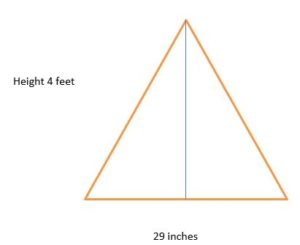This example, like the one above, entails more than simply adding like and identical measurement units like inches or feet. This example has the dissimilar measurement units of feet and inches. So, you must convert all measurement units to the same measurement unit before you can accurately perform the calculation.

In this example, you must convert all the measurements into either inches or feet.

For the purpose of this example, we will convert and use only feet, although you could also convert and use only inches as well unless your TEAS examination may require that you use inches only. Because the length of the base of the triangle is in inches, you will only have to convert the measurement of the height of the triangle into inches as follows, using ratio and proportion.

• 12 inches : 1 foot = x inches : 4 feet
• x = 12 x 4
• x = 48 inches

The height of this triangle is 48 inches so you can now calculate the area of this triangle using inches as the only unit of measurement because the feet unit of measurement was converted to the inches unit of measurement.

• Area = bh/2
• Area = 29 x 48/2
• Area = 1392/2
• Area = 696 square inches
• Answer - The area of this triangle is 696 square inches.

### Circles

Circles are very different from rectangles, squares and triangles.

Below is a list of the many differences among circles and rectangles, squares and triangles:

• Rectangles and squares are quadrilaterals, circles are not. Circles do not have sides.
• Rectangles and squares are four sided shapes, circles are not. Circles do not have sides.
• Rectangles and squares are a special kind of rhombus, circles are not. Circles are like eclipses and spheres.
• Rectangles, squares and triangles are polygons, circles are not. Circles do not have sides and they do not have multiple sides.
• Rectangles and squares have opposite sides that are equal to each other in terms of their length, circles do not have sides.
• Rectangles and squares have adjacent sides that are perpendicular to each other, circles do not have sides or perpendicular lines.
• Rectangles and squares have four sides and four right angles, each of which is right angles of 90 degrees.
• Rectangles and squares have a total of 360 degrees when all four of their interior right angles are added together and combined. Circles also have 360 degrees but not because all four of their interior right angles are added together and combined for 360 degrees; circles do not have interior right angles.
• Triangles have a total of 180 degrees when all three of their interior angles are added together and combined. Circles have 360 degrees but not because all three of their interior angles are added together and combined.
• Rectangles, squares and triangles have a length and a height, circles do no. Circles have other measurements like diameter, circumference and radii.
• Rectangles, squares and triangles can have perimeters that can be measured and calculated, circles do not. Circles have a circumference and not a perimeter.

Circles, as two dimensional shapes, are:

• Very uniform and symmetrical in terms of their shape and geometric properties. For example, a circle's radius and its length remain the same regardless of where the radius ends on the edge of the circle.
• Shapes that have 360 degrees

Some of the special terms and terminology that you should be knowledgeable about are:Circle with circumference (C) in black, diameter (D) in cyan, radius (R) in red, and center or origin (O) in magenta.

Circumference: The distance around the circle, as shown in the picture above. Circumferences for circles are the correlate of perimeters for squares, triangles, rectangles and other angular shapes like parallelograms, for example.

Circle's center: The point that is precisely in the middle of the circle from which lines of equal length can be drawn to one side (radius) or extended to both sides of the circle (diameter).

Diameter: The length of a straight line that passes through the center of the circle and extends in both directions to each side of the circle, as shown in the picture above. The diameter of a circle is two times the length of the same circle's radius. The diameter is a special kind of chord which will be defined and described below.

Radius: The length of a straight line that begins at the center of the circle and extends in one direction to any one side of the circle. The radius of a circle is ½ of the length of the same circle's diameter. Each and every radius on the same circle is equal in terms of its length regardless of where the end point of the straight line is on the circle, provided all lines are straight and all radii begin in the precise center of the circle.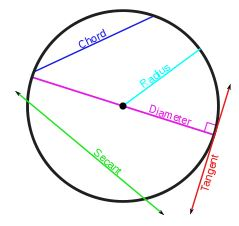Chord: A straight line that touches two points on the circle. This line does not have to pass through the center of the circle, like the diameter does. The diameter of a circle is a special kind of chord that must pass through the center of the circle. All other chords of the same circle can vary in terms of their positions and their lengths, as shown in the picture above.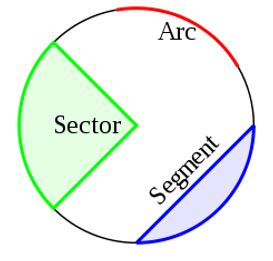Arc: An arc is any arc like continuous line that is part of a circle and its outer border, as shown in the picture above.

Segment: Any part or section of a circle that is outlined with one straight line that extends to two sides of the circle and an arc.

Sector: A portion or part of a circle that is outlined by two radii (pleural of radius) that begin in the center of the circle and an arc, as shown in the picture above.

#### The Concept of Pi

It is important, at this point of your TEAS review, that you know about and have an understanding of what is called pi.

In addition to being a Greek letter, pi has mathematical and geometric meaning and importance. Pi is an irrational number, like the square root of a number and in contrast to a rational number and pi is a mathematical constant that goes ∞ (infinity) unless it is rounded off. Pi is constant because the value of pi never changes

So, simply summarized, pi is:

• A mathematical constant that never changes and one that does not vary despite the changing of a circle's size. Pi always remains the same.
• An irrational number as contrasted to a rational number
• A value that goes on to infinity
• pi has a ratio relationship with the circumference of a circle
• Mathematically equal to pi is to 3.14159 rounded off because, as stated above, pi goes to infinity so it has to be rounded off.

#### Performing Geometric Calculations with Circles: Calculating the Circumference of a Circle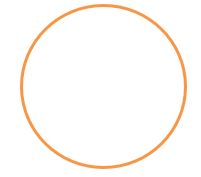As stated previously above, the circumference of a circle is analogous to the perimeter of a rectangle, square and triangle. The circumference of the circle above is outlined in orange.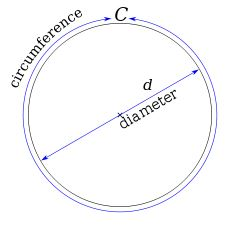The circumference of a circle is slightly more than three times as long as its diameter. The exact ratio is called π.

Because of the relationship of pi to the circumference of a circle, the geometric formula for the calculation of the circumference of a circle is:

• Circumference of a Circle = π x Diameter or 2 x π x Radius

OR

• π d or 2 π r

In order to calculate the circumference of the circle, you will have to know the value of pi, which is 3.14159, and the radius of the circle which is R.

As you calculate the circumferences of circles, the only information that you will need to get from the TEAS examination question is the radius of the circle because pi is a never changing mathematical constant of 3.14159.

Now, here are some examples of calculating the circumference of a circle.

Example 1:

What is the circumference of a circle that has a diameter of 4 cm?

• Circumference = π d
• Circumference = 3.14159 x 4 cm
• Circumference = 12.56636 cm
• Answer - The circumference of this circle is 12.56636 cm which can be rounded off to the nearest hundredth as 12.57 cm.

----------

Example 2:

What is the circumference of a circle that has a diameter of 6 inches?

• Circumference = π d
• Circumference = 3.14159 x 6 inches
• Circumference = 18.84954 inches
• Answer - The circumference of this circle is 18.84954 inches which can be rounded off to the nearest hundredth as 18.85 inches.

----------

Example 3:

What is the circumference of a circle that has a radius of 8 inches?

This problem, or calculation, is different from the two examples above because the information that is given to you in this question is that the radius is 8 inches you were not given the measurement of the diameter as you were given in the two examples above.

So, you have to now double the length of the radius from 8 inches to 16 inches because the diameter of a circle is 2 times the radius and the rule for calculating the circumference of a circle is π d or π x d and not π x r.

• Diameter = Radius x 2
• Diameter = 8 x 2 = 16
• Diameter = 16 inches

Now, you can calculate the circumference of the circle.

• Circumference = π d
• Circumference = 3.14159 x 16 inches
• Circumference = 50.26544 inches
• Answer - The circumference of this circle is 50.26544 inches which can be rounded off to the nearest hundredth as 50.27 inches.

----------

Example 4:

What is the circumference of a circle that has a radius of 7 cm?

This problem, or calculation, is similar to the problem immediately above because the information that is given to you in this question is that the radius is 7 cm and you were not given the measurement of the diameter.

So, again, you have to now double the length of the radius from 7 cm to 14 cm because the diameter of a circle is 2 times the radius and the rule for calculating the circumference of a circle is π d or π x d and not π x r.

• Diameter = Radius x 2
• Diameter = 7 x 2 = 14
• Diameter = 14 cm

Now, you can calculate the circumference of the circle.

• Circumference = π d
• Circumference = 3.14159 x 14 cm
• Circumference = 43.98226 cm
• Answer - The circumference of this circle is 43.98226 cm which can be rounded off to the nearest hundredth as 43.98 cm.

#### Performing Geometric Calculations with Circles: Calculating the Diameter of a CircleBased on the facts that the circumference of a circle is calculated with the formula π d and that the diameter is twice, or 2 times, the radius, you should be able to deduce that:

• Diameter = 2 r or 2 times the radius
• Radius = Diameter divided by 2
• Diameter = Circumference divided by π
• Circumference = π d
• C= π x 2r

Here are some examples of determining the diameter of a circle.

Example 1:

What is the diameter of a circle that has a radius of 23 cm?

• Diameter = 2 r
• d = 2 r
• d = 2 (23)
• d = 46 cm
• Answer - The diameter of a circle that has a radius of 23 cm is 46 cm which is twice the length of the radius of the circle.

----------

Example 2:

What is the diameter of a circle that has a radius of 5 feet?

• Diameter = 2 r
• d = 2 r
• d = 2 (5)
• d = 10 feet
• Answer - The diameter of a circle that has a radius of 23 cm5 feet is 10 feet which is twice the length of the radius of the circle.

----------

Example 3:

What is the diameter of a circle that has a radius of 4.6 cm?

• Diameter = 2 r
• d = 2 r
• d = 2 (4.6)
• d = 9.2 cm
• Answer - The diameter of a circle that has a radius of 4.6 cm is 9.2 cm which is twice the length of the radius of the circle.

----------

Example 4:

What is the diameter of a circle that has a radius of 4 meters?

• Diameter = 2 r
• d = 2 r
• d = 2 (4)
• d = 8 meters
• The diameter of a circle that has a radius of 4 meters is 8 meters which is twice the length of the radius of the circle.

----------

Example 5:

What is the diameter of a circle when ½ the radius of the circle is 6.6 cm?

This example is different from the 4 examples above because this question does not tell you the full length of the radius of the circle. Instead, this question gives you ½ the radius of the circle as 6.6 cm, so you have to determine the full length of the radius of the circle before you perform the typical calculation for the diameter of the circle.

This additional step is shown below.

Now, you can calculate the diameter of the circle because you have the measurement of the radius.

• Diameter = 2 r
• d = 2 r
• d = 2 (13.2)
• d = 26.4 cm
• Answer - The diameter of a circle that has a radius of 13.2 cm is 26.4 cm which is twice the length of the radius of the circle.

#### Performing Geometric Calculations with Circles: Calculating the Diameter of a Circle Knowing the Circumference

In addition to being able to calculate the diameter of a circle when you know the radius of a circle, you can also calculate the diameter of a circle when you know the circumference of the circle, because as stated previously, the circumference of a circle is based on pi (π) which is a mathematical constant that never changes in value.

So, if the rule for the circumference of a circle is:

Circumference = π d

And, based on the fact that π is always 3.14159 regardless of the size of the circle, you should be able to deduce and understand that you can also use this rule to determine the circumference of a circle:

Circumference = 3.14159 x d

Stay with us here, there is only one other deduction that we want you to understand.

Because you now know that π is ALWAYS 3.14159 and you are told what the circumference is, all you have to now determine is the diameter of the circle.

Circumference that you are given in the problem will be equal to 3.14159, which is ALWAYS the same, multiplied by x Diameter which you have to calculate

The diameter is the only unknown is this calculation.

Here is an example:

What is the diameter of a circle that has a circumference of 45 cm?

In this problem you are asked to calculate the diameter of a circle, the only unknown in this word problem. You were told that the circumference of this particular circle is 45 cm and you know that π is ALWAYS 3.14159, so:

• Diameter = Circumference Divided by 3.14159
• d = 45 ÷ 3.14159
• d = 14.323 cm which can be rounded off to the nearest hundredth as 14.32 cm.
• Answer - The diameter of a circle that has a circumference of 45 cm is 14.32 cm.

In order to check this example and to insure that your calculations were correct and accurate, you can calculate the circumference of the circle by using the diameter of 14.32 cm, as shown below:

• Circumference = π d
• C = 3.14159 x 14.32
• C = 44.98 cm which can be rounded off to 45 cm

PLEASE NOTE: The circumference of 45 cm was the number that you were originally given in this word problem, so when you proofed your calculation with the reverse calculation, you are certain that you accurately and precisely determined the diameter of the circle as 14.32 cm.

Here are some more examples of calculating the diameter of a circle when you are given the circumference of the circle.

Example 1:

What is the diameter of a circle that has a circumference of 16 inches?

• Diameter = Circumference Divided by 3.14159
• d = 16 ÷ 3.14159
• d = 5.0929 inches which can be rounded off to the nearest hundredth as 5.09 inches
• Answer - The diameter of a circle that has a circumference of 16 inches is 5.09 inches.

----------

Example 2:

What is the diameter of a circle that has a circumference of 88 cm?

• Diameter = Circumference Divided by 3.14159
• d = 88 ÷ 3.14159
• d = 28.0112 cm which can be rounded off to the nearest hundredth as 28.01 cm
• Answer - The diameter of a circle that has a circumference of 88 cm is 28.01 cm.

----------

Example 3:

What is the diameter of a circle that has a circumference of 88 cm?

• Diameter = Circumference Divided by 3.14159
• d = 88 ÷ 3.14159
• d = 28.0112 cm which can be rounded off to the nearest hundredth as 28.01 cm
• Answer - The diameter of a circle that has a circumference of 88 cm is 28.01 cm.

----------

Example 4:

What is the diameter of a circle that has a circumference of 3.76 meters?

• Diameter = Circumference Divided by 3.14159
• d = 3.76 ÷ 3.14159
• d = 1.1968 meters which can be rounded off to the nearest hundredth as 1.20 meters or 1.2 meters
• Answer - The diameter of a circle that has a circumference of 3.76 meters is 1.2 meters

----------

Example 5:

What is the diameter of a circle that has a circumference of 6.98 feet?

• Diameter = Circumference Divided by 3.14159
• d = 6.98 feet ÷ 3.14159
• d = 2.2218 feet which can be rounded off to the nearest hundredth as 2.22 feet
• Answer - The diameter of a circle that has a circumference of 6.98 feet is 2.22 feet.

#### Performing Geometric Calculations with Circles: Calculating the Radius of a Circle Knowing the Diameter of a CircleThe rule to calculate the radius of a circle is:

• Radius = Diameter divided by 2

OR

• R = d/2

Here are some examples of determining the radius of a circle.

Example 1:

What is the radius of a circle when the diameter of the circle is 42.3 cm?

• R = d/2
• R = 42.3 /2
• R = 21.5 cm
• Answer - The radius of a circle that has a diameter of 42.3 cm is 21.5 cm which is one half the length of the diameter of the circle.

----------

The rule to calculate the radius of a circle is:

Radius = Diameter divided by 2 or

R = d/2

Here are some examples of determining the radius of a circle.

Example 2:

What is the radius of a circle when the diameter of the circle is 42.3 cm?

• R = d/2
• R = 42.3 /2
• R = 21.5 cm
• Answer - The radius of a circle that has a diameter of 42.3 cm is 21.5 cm which is one half the length of the diameter of the circle.

----------

Example 3:

What is the radius of a circle when the diameter of the circle is 24.9 cm?

• R = d/2
• R - 24.9/2
• R = 12.45 cm
• Answer - The radius of a circle that has a diameter of 24.9 cm is 12.45 cm which is one half the length of the diameter of the circle.

-----------

Example 4:

What is the radius of a circle when the diameter of the circle is 32 yards?

• R = d/2
• R = 32/2
• R = 16 yards
• Answer - The radius of a circle that has a diameter of 32 yards is 16 yards which is one half the length of the diameter of the circle.

-----------

Example 5:

What is the 1/2 of radius of a circle when the diameter of the circle is 33 cm?

This example is different from the four above in that this question does NOT ask for the full radius of the circle, instead, it asks you to calculate 1/2 of the radius. In order to calculate 1/2 of the radius of this circle, first determine the length of the full radius as you have done in the four examples above this and then calculate 1/2 of this length, as below.

• R = d/2
• R = 33/2
• R = 16.5 cm

The full radius of a circle that has a diameter of 33 cm is 16.5 cm because the radius of a circle is ½ the diameter of the circle.

Now, you can calculate ½ of the radius of this circle, as below.

• 1/2r = x
• x = 1/2 x 8.25 cm
• Answer - 1/2 of the radius of this circle is 8.25 cm and the full radius of this circle is 16.5 because the radius is ½ of the diameter of this circle which has a diameter of 33 cm.

#### Performing Geometric Calculations with Circles: Calculating the Radius of a Circle Knowing the Circumference

In addition to being able to calculate the diameter of a circle when you the circumference of the circle, because as stated previously, the circumference of a circle is based on pi (π) which is a mathematical constant that never changes in value.

The rule for the circumference of a circle is:

• Circumference = π d

Because the diameter of a circle is twice the radius of the circle and the radius is ½ the diameter, an alternative formula or rule to calculate the circumference is:

• Circumference = π x 2r

So, based on the fact that π is always 3.14159 regardless of the size of the circle, you should be able to deduce and understand that you can also use this rule to determine the circumference of a circle:

• Circumference = 3.14159 x 2r

Stay with us here, there is only one other deduction that we want you to understand.

Because you now know that π is ALWAYS 3.14159 and you are told what the circumference is, all you have to now determine is the diameter of the circle and then divide that diameter by 2 to determine the radius of the circle.

The radius is the only unknown is this calculation.

Here is an example:

What is the radius of a circle that has a circumference of 45 cm?

In this problem you are asked to calculate the radius of a circle, the only unknown in this word problem. You were told that the circumference of this particular circle is 45 cm and you know that π is ALWAYS 3.14159, so:

• 2 r = Circumference Divided by 3.14159
• 2 r = 45 / 3.14159
• 2 r = 14.323 cm
• r = 7.1615 cm which can be rounded off to the nearest hundredth as 7.16 cm
• Answer - The radius of a circle that has a circumference of 45 cm is 7.16 cm.

Here are some more examples of calculating the radius of a circle when you are given the circumference of the circle.

Example 1:

What is the radius of a circle that has a circumference of 16 inches?

• 2 r = Circumference Divided by 3.14159
• 2 r = 16 ÷ 3.14159
• 2 r = 5.0929 cm
• r = 2.5464 cm which can be rounded off to the nearest hundredth as 2.55 cm
• Answer - The radius of a circle that has a circumference of 16 inches is 5.09 inches.

----------

Example 2:

What is the radius of a circle that has a circumference of 88 cm?

• 2 r = Circumference Divided by 3.14159
• 2 r = 88 ÷ 3.14159
• 2 r = 28.0112 cm
• r = 14.0056 which can be rounded off to the nearest hundredth as 14 cm
• Answer - The radius of a circle that has a circumference of 88 cm is 14 cm.

----------

Example 3:

What is the radius of a circle that has a circumference of 20 yards?

• 2 r = Circumference Divided by 3.14159
• 2 r = 20 ÷ 3.14159
• 2r = 6.3662 yards
• r = 3.1831 which can be rounded off to the nearest hundredth as 3.18 yards
• Answer - The radius of a circle that has a circumference of 20 yards is 3.18 yards.

----------

Example 4:

What is the radius of a circle that has a circumference of 6.98 feet?

2 r = Circumference Divided by 3.14159

2 r = 6.98 feet ÷ 3.14159

2 r = 2.2218 feet

r = 1.1109 which can be rounded off to the nearest hundredth as 1.11 feet

Answer - The radius of a circle that has a circumference of 6.98 feet is 1.11 feet.

#### Squared

You will have to know about square roots and how to calculate them when you are asked to perform calculations relating to circles and some other arithmetic and mathematical calculations.

Numbers are squared by multiplying the number or integer by itself. For example, if you have to square 6, the calculation is 6 x 6 or 36. The squares of numbers are written with a 2 above the line of the text where the number is.

For example, 62 is 6 squared.

The number m is a square number if and only if one can compose a square of m equal (lesser) squares: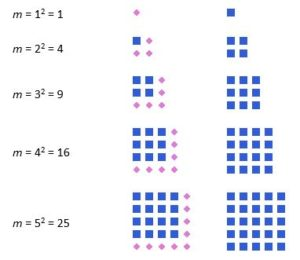In other words, the square of a number is written as:

• 62
• 72
• 122
• 112

Some of the characteristics of squared numbers or integers include:

• The squares of all even numbers and integers like 2, 4, 6, 8, 10… are always even numbers
• The squares of all odd numbers and integers like 3, 5, 7, 9, 11… are always odd numbers
• All even squares can be evenly divided by 4. For example, the square of 16 is 256 and 256 is evenly divisible by 4; the square of 22 is 484 and 484 is evenly divisible by 4; and the square of 492 is 242,064 and 242,064 is evenly divisible by 4.

The characteristics of squared numbers or integers, as listed above, are highly useful to you so that you can quickly and readily check your calculations when you are asked to square a number.

For example:

If you are asked to square the number 21 and you get an answer that is even, you should immediately know that your answer is incorrect because 21 is an odd number and all squares of all odd numbers and integers are odd numbers and not even numbers.

If you are asked to square the number 28 and you get an answer that is odd, you should immediately know that your answer is incorrect because 28 is an even number and all squares of all even numbers and integers are even numbers and not odd numbers AND

If you are asked to square the number 28 and you get an answer that is not evenly divisible by 4, you should immediately know that your answer is incorrect because 28 is an even number and all squares of all even numbers and integers are evenly divisible by 4 WITHOUT anything left over and WITHOUT any decimal places after the whole number or integer.

Here are some examples of determining and calculating the squares of numbers.

Example 1:

What is the square of 3?

• Square = Number x Number
• Square = n x
• Square = 3 x 3
• Square = 9

Note that the answer to this problem is an odd number and the number that was squared is also an odd number, so this answer is likely correct. However, if you got an even number as the square of that odd number, you should immediately know that this answer is not correct because all odd numbers and integers squared will yield an odd number and not an even number.

• Answer - The square of 3 is 9.

-----------

Example 2:

What is the square of 7?

• Square = Number x Number
• Square = n x n
• Square = 7 x 7
• Square = 49

Note that the answer to this problem is an odd number and the number that was squared is also an odd number, so this answer is likely correct. However, if you got an even number as the square of the number, you should immediately know that this answer is not correct because all odd numbers and integers squared will yield an odd number and not an even number.

• Answer - The square of 7 is 49.

----------

Example 3:

What is the square of 4.4?

• Square = Number x Number
• Square = n x n
• Square = 4.4 x 4.4
• Square = 19.36

Note that the answer to this problem is an even number and the number that was squared is also an even number, so this answer is likely correct. However, if you got an odd number as the square of the number, you should immediately know that this answer is not correct because all even numbers and integers squared will yield an even number and not an odd number.

• Answer - The square of 4.4 is 19.36.

-----------

Example 4:

What is the square of 1?

• Square = Number x Number
• Square = n x n
• Square = 1 x 1
• Square = 1

Note that, again, the answer to this problem is an odd number and the number that was squared is also an odd number, so this answer is likely correct. However, if you got an even number as the square of the number, you should immediately know that this answer is not correct because all odd numbers and integers squared will yield an odd number and not an even number.

• Answer - The square of 1 is 1.

-----------

Example 5:

What is the square of - 100?

• Square = Number x Number
• Square = n x n
• Square = -100 x -100
• Square = + 10,000 or 10,000 (A negative number multiplied by a negative number is a positive number.

Note that the answer to this problem is an even number and the number that was squared is also an even number, so this answer is likely correct. However, if you got an odd number as the square of the number, you should immediately know that this answer is not correct because all even numbers and integers squared will yield an even number and not an odd number.

• Answer: The square of – 10 is 10,000

#### Square RootsThe mathematical expression "The (principal) square root of x"

The sign above that looks a little similar to the division symbol is used to indicate square root. This symbol is referred to as a radical.

The square root of the number is the reverse calculation of calculating a square of a number. In other words, the square of a number is calculated by multiplying the number or integer by itself and the square root of a number is calculated by dividing (the reverse operation for multiplication) the number or integer by itself.

Some of the characteristics of the square roots of numbers or integers include:

• Because the squares of all even numbers and integers like 2, 4, 6, 8, 10… are always even numbers, the square roots of all even numbers and integers are also even numbers.
• Because the squares of all odd numbers and integers like 3, 5, 7, 9, 11… are always odd numbers, the square roots of all odd numbers and integers are also odd numbers.
• Because all even squares can be evenly divided by 4, the square roots of even numbers can also be evenly divisible by 4.

The characteristics of square roots, as listed above, are highly useful to you so that you can quickly and readily check your calculations when you are asked to calculate the square root of a number.

For example:

If you are asked to calculate the square root of the number 33 and you get an answer that is even, you should immediately know that your answer is incorrect because 33 is an odd number and all square roots of all odd numbers and integers are odd numbers and not even numbers.

If you are asked to calculate the square root of the number 28 and you get an answer that is odd, you should immediately know that your answer is incorrect because 28 is an even number and all square roots of all even numbers and integers are even numbers and not odd numbers AND

If you are asked to calculate the square root of the number 28 and you get an answer that is not evenly divisible by 4, you should immediately know that your answer is incorrect because 28 is an even number and all square roots of all even numbers and integers are evenly divisible by 4 WITHOUT anything left over and WITHOUT any decimal places after the whole number or integer.

Here are some examples of determining and calculating the square roots of numbers.

Example 1:

What is the square root of 81?

• Square Root = Number / Number
• Square Root = 9 which can be determined by using the square root function on your calculator or relying on your memory that 9 x 9 = 81.
• Square Root = 9

Note that the square root answer to this problem is an odd number and the number that you used to find the square root of is also an odd number, so this answer is likely correct. However, if you got an even number as the square root of the number, you should immediately know that this answer is not correct because all odd numbers and integers will yield an odd number and not an even number for its square root.

• Answer - The square root of 81 is 9.

----------

Example 2:

What is the square root of 67?

Square Root = Number ÷ Number

Square Root = 8.185…5 which can be determined by using the square root function on your calculator. The square root of 8.185…5 was written with the 8.185 as shown on the calculator but there were several other digits between those numbers and the last 5 that were shown on the calculator and indicated as … to avoid confusion and based on the fact that these digits were not necessary to demonstrate the fact that the square root is an odd number ending in 5.

Note that the square root answer to this problem is an odd number and the number that you used to find the square root of is also an odd number, so this answer is likely correct. However, if you got an even number as the square root of the number, you should immediately know that this answer is not correct because all odd numbers and integers will yield an odd number and not an even number for its square root.

• Answer - The square root of 67 is 8.185 rounded off to the nearest thousandth.

-----------

Example 3:

What is the square root of 144?

Square Root = Number ÷ Number

Square Root = 12 which can be determined by using the square root function on your calculator or relying on your memory that 12 x 12 = 144.

Note that the square root answer to this problem is an even number and the number that you used to find the square root of is also an even number, so this answer is likely correct. However, if you got an odd number as the square root of the number, you should immediately know that this answer is not correct because all even numbers and integers will yield an even and not an odd number for its square root.

• Answer - The square root of 144 is 12.

----------

Example 4:

What is the square root of 3136?

Square Root = Number ÷ Number

Square Root = 56 which can be determined by using the square root function on your calculator.

Note, again, that the square root answer to this problem is an even number and the number that you used to find the square root of is also an even number, so this answer is likely correct. However, if you got an odd number as the square root of the number, you should immediately know that this answer is not correct because all even numbers and integers will yield an even and not an odd number for its square root.

• Answer - The square root of 3136 is 56.

----------

Example 5:

What is the square root of 1?

Square Root = Number ÷ Number

Square Root = 1 which can be determined by using the square root function on your calculator or by recalling that 1 x 1 = 1.

Note that the square root answer to this problem is an odd number and the number that you used to find the square root of is also an odd number, so this answer is likely correct. However, if you got an even number as the square root of the number, you should immediately know that this answer is not correct because all odd numbers and integers will yield an odd and not an even number for its square root.

• Answer - The square root of 1 is 1.

#### Performing Geometric Calculations with Circles: Calculating the Area of a Circle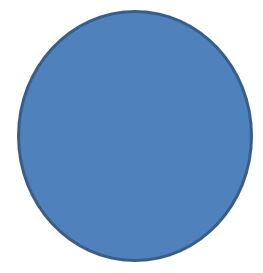Like the need for and the desire to calculate and know the areas of rectangles, squares and triangles, there are many everyday situations when you will want to find and calculate the area of a circle.

As you know, areas of shapes, including circles, of rectangles, squares and triangles, are very different from the circumference of circles and the perimeters of rectangles, squares and triangles. Areas are not a border around a shape, areas are the entire interior surface of the shape inside its border.

The figures below show the circumference of a circle and the perimeters of a rectangle, a square and a triangle outlined in orange. Circumferences and perimeters are often determined and calculated to find out and determine the lengths of things like fences, trims and borders.The figures below show the areas of a circle, a rectangle, a square and a triangle colored in the color black. The areas of shapes are often determined and calculated to find out and determine the number of square cm, square inches, square feet, square meters and other units of measurement that are needed for things like carpet, rugs, lawns, concrete areas and other flat circular structures.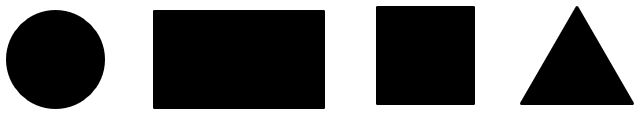The formula for the area of a circle, unlike the formulas for other shapes like rectangles, squares and triangles is based on π (pi) and it is:

Area of a Circle = π r 2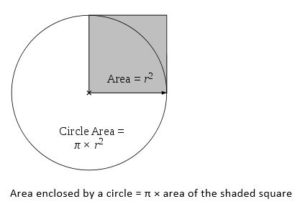As you can see in the circle above r 2 is the part of the circle that is shaded in gray. Below is the procedure for calculating the area of a circle.

• Area of a Circle = π r 2

If the radius of the circle is 4 cm, you would calculate the area of the circle as:

• Area of the Circle = 3.14159 x 4 2
• Area of the Circle = 3.14159 x 16
• Area of the Circle = 50.2654 square cm which can be rounded off to the nearest hundredth as 50.27 square cm.

Let's now practice calculations to find the area of a circle.

Example 1:

What is the area of a circle that has a radius of 12 cm?

• Area of a Circle = π r 2
• a = π r 2
• a = 3.14159 x r 2
• a = 3.14159 x 122
• a = 3.14159 x 144
• a = 452.3889 square cm which can be rounded off to the nearest hundredth as 452.38 square cm
• Answer - The area of a circle that has a radius of 12 cm is square 452.38 square cm.

----------

Example 2:

What is the area of a circle that has a radius of 6 inches?

• Area of a Circle = π r 2
• a = π r 2
• a = 3.14159 x r 2
• a = 3.14159 x 6 2
• a = 3.14159 x 36
• a = 113.0972 square inches which can be rounded off to the nearest hundredth as 113.10 or 113.1 inches
• Answer - The area of a circle that has a radius of 56 inches is 113.1 square inches.

----------

Example 3:

What is the area of a circle that has a radius of 7 meters?

• Area of a Circle = π r 2
• a = π r 2
• a = 3.14159 x r 2
• a = 3.14159 x 72
• a = 3.14159 x 49
• a = 153.9379 square meters which can be rounded off to the nearest hundredth as 153.94 square meters
• Answer: The area of a circle that has a radius of 7 meters is 153.94 square meters.

#### Performing Geometric Calculations with Circles: Calculating the Radius of a Circle Knowing the Area of the Circle

Similar to the calculations of the diameter of a circle based on the circumference and radius of a circle and the calculations of the radius of a circle based on the circumference and diameter of a circle, you can also calculate the radius of a circle knowing the area of the circle because pi is ALWAYS a mathematical constant that NEVER changes in terms of its value which is 3.14159.

Since the formula for the area of a circle is:

Area of a circle = π r 2

and because, as you should now know that, π will ALWAYS be 3.14159, you could write the formula for the area of a circle simply as:

Area of a circle = 3.14159 x r 2.

When you are asked to calculate the area of a circle on your TEAS examination, you will be given only one piece of information in the question to perform this calculation. You will be given the radius of the circle. You will NOT be given any information about π because you should have memorized and know that π is ALWAYS 3.14159 regardless of the size of the circle so your TEAS examination will not give you this information.

So, based on your knowledge of π and knowing that the TEAS examination question will give you information about the radius, the only thing left for you to calculate and determine is the area of the circle.

Similarly, if the TEAS examination question tells you what the area of a circle is, you should be able to accurately calculate the radius of the circle, again, because π is ALWAYS 3.14159 regardless of the size of the circle.

Here is an example of calculating the radius of the circle based on the area of the circle.

What is the radius of a circle when the area of that circle is 46 square inches?

• Area of a circle = 3.14159 x r 2.
• r 2 = Area of a circle divided by 3.14159 (π)
• r 2 = 46 inches / 3.14159
• r 2 = 14.6422
• r = square root of 14.6422
• r = 2.8265…1
• r = 2.83 inches rounded off to the nearest hundredth
• Answer - The radius of a circle that has an area of 46 square inches is 2.83 inches.

Here are more examples of finding the radius of a circle when you are given the area of that same circle.

Example 1:

What is the radius of a circle when the area of that circle is 24 sq. feet

• Area of a circle = 3.14159 x r 2.
• r 2 = Area of a circle divided by 3.14159 (π)
• r 2 = 24 ft. / 3.14159
• r 2 = 7.6394…7
• r = square root of 7.6394…7
• r = 2.7639 or 2.76 cm rounded off to the nearest hundredth
• r = 2.76 feet rounded off to the nearest hundredth
• Answer - The radius of a circle that has an area of 24 sq. feet is 2.76 feet.

You can check this answer by calculating the area of the circle based on the radius of the circle that you just calculated. If, when you calculate the area of the circle, you get the answer for the area that is the same that was given to you in the original question, your calculation of the radius was correct. If, however, you calculate the area of the circle, and you do not get the answer for the area that is the same that was given to you in the original question, your calculation of the area of the circle and/or your calculation of the radius was incorrect.

• Area of a circle = 3.14159 x r 2
• a = 3.14159 x 2.76 2
• a = 3.14159 x 7.6176
• a = 23.93

The area of a circle with a radius of 2.76 feet is 23.93, which rounded off to the nearest foot is 24 sq. feet which is precisely what question stated as the area of the circle for which you were asked to calculate the radius.

----------

Example 2:

What is the radius of a circle when the area of that circle is 36 sq. cm?

• Area of a circle = 3.14159 x r 2.
• r 2 = Area of a circle divided by 3.14159 (π)
• r 2 = 36 cm / 3.14159
• r 2 = 11.4591…5
• r = square root of 11.4591…5
• r = 3.3851 cm
• r = 3.39 cm rounded off to the nearest hundredth
• Answer - The radius of a circle that has an area of 36 sq. cm is 3.39 cm

You can check this answer by calculating the area of the circle based on the radius of the circle that you just calculated. If, when you calculate the area of the circle, you get the answer for the area that is the same that was given to you in the original question, your calculation of the radius was correct. If, however, you calculate the area of the circle, and you do not get the answer for the area that is the same that was given to you in the original question, your calculation of the area of the circle and/or your calculation of the radius was incorrect.

• Area of a circle = 3.14159 x r 2
• a = 3.14159 x 3.39 2
• a = 3.14159 x 11.4921
• a = 23.9336.10

The area of a circle with a radius of 3.39 feet is 23.93, which rounded off to the nearest foot is 24 sq. feet which is precisely what question stated as the area of the circle for which you were asked to calculate the radius.

----------

Example 3:

What is the radius of a circle when the area of that circle is 77 meters

• Area of a circle = 3.14159 x r 2.
• r 2 = Area of a circle divided by 3.14159 (π)
• r 2 = 77 meters / 3.14159
• r 2 = 24.5098…9
• r = square root of 24.5098…9
• r = 4.9507
• r = 4.96 meters rounded off to the nearest hundredth
• Answer - The radius of a circle that has an area of 77 sq. meters is 4.96 meters.

You can check this answer by calculating the area of the circle based on the radius of the circle that you just calculated. If, when you calculate the area of the circle, you get the answer for the area that is the same that was given to you in the original question, your calculation of the radius was correct. If, however, you calculate the area of the circle, and you do not get the answer for the area that is the same that was given to you in the original question, your calculation of the area of the circle and/or your calculation of the radius was incorrect.

• Area of a circle = 3.14159 x r 2
• a = 3.14159 x 4.96 2
• a = 3.14159 x 24.6061
• a = 77.2811

The area of a circle with a radius of 4.96 meters is 77.2811, which rounded off to the nearest meter is 77 sq. meters which is precisely what question stated as the area of the circle for which you were asked to calculate the radius.

### Parallelograms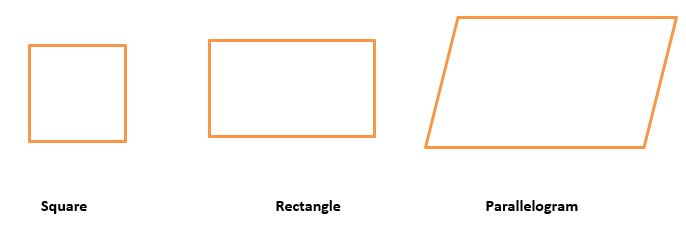A parallelogram, as shown above, is an interesting shape that has some similarities and commonalities with other shapes such as a rectangle and a square.

With a simple inspection of the parallelogram above, you can see that a parallelogram shares similarities and commonalities with a square. Both a parallelogram and a square:

• Have four sides.
• Have two pairs of opposite sides that are parallel to each other.
• Have four interior angles, one in each corner.
• Have four interior angles that total 360 degrees.
• Have two pairs of opposite interior angles that are equal and congruent with each other.

Looking at the above similarities and commonalities that a parallelogram shares with a square, you may be able to visually see that there are some major differences between a parallelogram and a square, For example:

• Although both a square and a parallelogram have four sides, a square has four sides that are equal in terms of length and a parallelogram has four sides that do NOT have to be equal in terms of length, although at times they could and can be equal in terms of length.
• Although both a square and a parallelogram have four interior angles, one in each corner, the square has four equal and congruent interior angles of 90 degrees each and the parallelogram has two pairs of opposite angles are equal and congruent to each other and not four equal and congruent angles but not four equal and congruent angles of 90 degrees each.
• Although both a square and a parallelogram have two pairs of opposite interior angles that are equal and congruent with each other, the square has all four interior angles that are equal and congruent with each other and the parallelogram only has only two pairs of opposite interior angles that are equal and congruent with each other.
• Parallelograms have NO 90 degree right angles or perpendicular lines like a square does.

You should also be able to see the similarities and commonalities that a parallelogram shares with a rectangle. Both a parallelogram and a rectangle:

• Have four sides.
• Have two pairs of opposite sides that are parallel to each other.
• Have four interior angles, one in each corner.
• Have four interior angles that total 360 degrees.
• Have two pairs of opposite interior angles that are equal and congruent with each other.

Looking at the above similarities and commonalities that a parallelogram shares with a rectangle, there are some major differences between a parallelogram and a rectangle,

For example:

• Although both a rectangle and a parallelogram have four sides, a rectangle has four sides that have two opposite sides are equal in terms of length and a parallelogram has four sides that do NOT have to have any sides that are equal in terms of length, although at times they can be equal in terms of length.
• Although both a rectangle and a parallelogram have four interior angles, one in each corner, the rectangle has four equal and congruent interior angles of 90 degrees each and the parallelogram has two pairs of opposite angles that are equal and congruent to each other but not four equal and congruent angles of 90 degrees each.
• Parallelograms have NO 90 degree right angles or perpendicular lines like a square does.

So, based on the above commonalities, similarities and difference among a parallelogram, square and rectangle, some of the characteristics that they all have in common is the fact that all three have:

• Four sides.
• Two pairs of opposite sides that are parallel to each other.
• Have four interior angles, one in each corner.
• Have four interior angles that total 360 degrees.
• Have two pairs of opposite interior angles that are equal and congruent with each other.

More commonalities, similarities and difference among a parallelogram, square and rectangle will be explored and discussed below.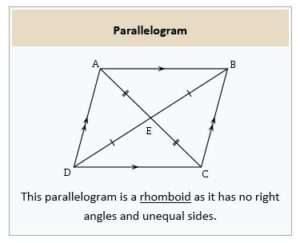Simply defined, a parallelogram is a two dimensional quadrilateral with 4 sides that has two pairs of opposite:

• Sides that are equal in terms of length. See the lines in the picture above that shows lines AB and DC as well as lines AD and BC; these two pairs of lines are equal in terms of length.
• Sides that are parallel to each other. See the lines in the picture above that shows lines AB and DC as well as lines AD and BC; these two pairs of lines are parallel to each other.
• Opposite angles that are equal and congruent to each other.
• Angles that are supplementary to each other.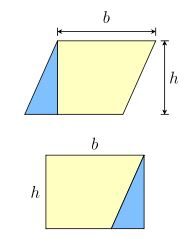A parallelogram can be rearranged into a rectangle with the same area.

The other defining characteristics of a parallelogram are that a parallelogram has:

• The ability to form a rectangle of the precise area when it is rearranged as a rectangle, as shown in the picture above.
• Diagonals that bisect each other.
• Bisecting diagonals that create and divide the parallelogram into two pairs of congruent triangles. See the angles in the picture above that shows these two congruent pairs of triangles as AED and BEC as one opposite pair of congruent triangles and triangles AEB and DEC as the second pair of congruent triangles.
• Each diagonal creates and divides the parallelogram into two equal and congruent triangles. See these two triangles in the picture above that shows one of these two equal and congruent triangles as ABCE and the other as ADCE with the same base of AEC. These two equal and congruent triangles are seen in the lower left hand side of the parallelogram and the upper right side of the parallelogram that are formed by the one diagonal line labeled AEC which is also the base of both of these two congruent and identical triangles.
• A second diagonal creates and divides the parallelogram into another set or pair of two different, equal and congruent triangles. See these two triangles in the picture above that shows one of these two equal and congruent triangles as DABE and the other as BCDE with the same base of DEB. These two equal and congruent triangles are seen in the upper left hand side of the parallelogram and the lower right side of the parallelogram that are formed by the one diagonal line labeled DEB which is also the base of both of these two congruent and identical triangles.
• Two sets of adjacent, not opposite, angles that are NOT equal but, instead, supplementary to each other and, that, as supplementary angles, add up to 180 degrees.
• Four interior angles that total 360 degrees.

#### Performing Geometric Calculations with Parallelograms: Calculating the Perimeter of a Parallelogram

The formula for the perimeter of a parallelogram is identical to the formula for calculating the perimeter of a rectangle because both are quadrilateral or four sided figures that have 2 sets of parallel and equal sides in terms of their lengths so, the formula for the perimeter of a parallelogram is:

• Perimeter of a parallelogram = Side 1 + Side 2 + Side 3 + Side 4

OR

• Perimeter of a parallelogram = 2 x length of the base + 2 x the length of the height.

The similarity of a parallelogram and a rectangle is shown in the picture below.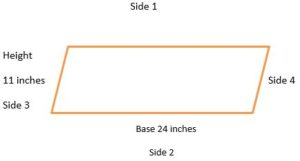Here is an example of calculating the perimeter of a parallelogram.

The parallelogram above has a base of 24 inches and a height of 11 inches. For the purpose of this exercise, we will call the top side of the parallelogram above as side 1, the lower side of this parallelogram as side 2, the left side of the parallelogram above as side 3 and the right side of this parallelogram as side 4 , as shown above.

• Perimeter of a parallelogram = Side 1 + Side 2 + Side 3 + Side 4
• Perimeter of a parallelogram = 24 + 24 + 11 + 11
• Perimeter of a parallelogram = 48 + 22
• Perimeter of a parallelogram = 70 inches
• x = 70 inches
• Answer - The perimeter of this parallelogram is 70 inches.

An alternative way to calculate the perimeter of the same parallelogram is:

• Perimeter of a parallelogram = 2 x length of the base + 2 x the length of the height
• Perimeter of a parallelogram = 2 (24) + 2(11)
• Perimeter of a parallelogram = 48 + 22
• Perimeter of a parallelogram = 70 inches
• x = 70 inches
• Answer - The perimeter of this parallelogram is 70 inches.

Now, here are some practice examples for calculating the perimeter of a parallelogram.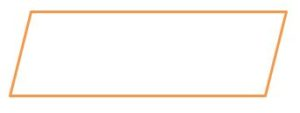Example 1:

What is the perimeter of a parallelogram that has a base of 32 cm and a height of 67 cm?

• Perimeter of a parallelogram = Base Length + Top Length + Right Side Height + Right Side Height
• Perimeter of a parallelogram = 32 + 32 + 67 + 67
• Perimeter of a parallelogram = 64 + 134
• Perimeter of a parallelogram = 198 cm
• x = 198 cm
• Answer: The perimeter of this parallelogram is 198 cm.

An alternative way to calculate the perimeter of the same parallelogram is:

• Perimeter of a parallelogram = 2 x Length of the Base + 2 x The Length of the Height
• Perimeter of a parallelogram = 2 (32) + 2(67)
• Perimeter of a parallelogram = 64 + 134
• Perimeter of a parallelogram = 198 cm
• x = 198 cm
• Answer - The perimeter of this parallelogram is 198 cm.

----------

Example 2:

What is the perimeter of a parallelogram that has a base of 9 feet and a height of 3 feet?

• Perimeter of a parallelogram = Base Length + Top Length + Right Side Height + Right Side Height
• Perimeter of a parallelogram = 9 + 9 + 3 + 3
• Perimeter of a parallelogram = 18 + 6
• Perimeter of a parallelogram = 24 feet
• x = 24 feet
• Answer - The perimeter of this parallelogram is 24 feet.

An alternative way to calculate the perimeter of the same parallelogram is:

• Perimeter of a parallelogram = 2 x Length of the Base + 2 x The Length of the Height
• Perimeter of a parallelogram = 2 (9) + 2(3)
• Perimeter of a parallelogram = 18 + 6
• Perimeter of a parallelogram = 24 feet
• x = 24 feet
• Answer - The perimeter of this parallelogram is 24 feet.

----------

Example 3:

What is the perimeter of a parallelogram that has a base of 9 feet km and a height of 3 feet?

• Perimeter of a parallelogram = Base Length + Top Length + Right Side Height + Right Side Height
• Perimeter of a parallelogram = 9 + 9 + 3 + 3
• Perimeter of a parallelogram = 18 + 6
• Perimeter of a parallelogram = 24 feet
• x = 24 feet
• Answer - The perimeter of this parallelogram is 24 feet.

An alternative way to calculate the perimeter of the same parallelogram is:

• Perimeter of a parallelogram = 2 x Length of the Base + 2 x The Length of the Height
• Perimeter of a parallelogram = 2 (9) + 2(3)
• Perimeter of a parallelogram = 18 + 6
• Perimeter of a parallelogram = 24 feet
• x = 24 feet
• Answer - The perimeter of this parallelogram is 24 feet.

#### Performing Geometric Calculations with Parallelograms: Calculating the Height of a Parallelogram Knowing the Perimeter and the Base of the Parallelogram

Very similar to what you should have already learned in the sections above, you can apply your knowledge of algebra and reverse operations or calculations to find the different variables in area and perimeter rules, knowing the area or perimeter of a shape.

Here is another example of finding an unknown variable that entails finding the height of a parallelogram when you are given the perimeter and the base of the parallelogram.

What is the height of a parallelogram that has a perimeter of 34 inches and a base of 11 inches?

• Perimeter of a parallelogram = Side 1 + Side 2 + Side 3 + Side 4

OR

• Perimeter of a parallelogram = 2 x length of the base + 2 x the length of the height.
• 34 = 2 (11) + 2(x)
• 34 = 22 + 2x
• 2x = 34 – 22
• 2x = 12
• x = 12 ÷ 2
• x = 6 inches
• Answer - The height of the parallelogram is 6 inches when the base is 11 inches and the perimeter of the parallelogram is 34 inches.

Here are some more practice examples:

Example 1:

What is the height of a parallelogram that has a perimeter of 22 km and a base of 4 km?

• Perimeter of a parallelogram = Side 1 + Side 2 + Side 3 + Side 4

OR

• Perimeter of a parallelogram = 2 x length of the base + 2 x the length of the height.
• 22 = 2 (4) + 2(x)
• 22 = 8 + 2x
• 2x = 22 – 8
• 2x = 14
• x = 14 ÷ 2
• x = 7 km
• Answer - The height of the parallelogram is 7 km when the base is 4 km and the perimeter of the parallelogram is 22 km.

----------

Example 2:

What is the height of a parallelogram that has a perimeter of 14.2 inches and a base of 4 inches?

• Perimeter of a parallelogram = Side 1 + Side 2 + Side 3 + Side 4

OR

• Perimeter of a parallelogram = 2 x length of the base + 2 x the length of the height.
• 14.2 = 2 (4) + 2(x)
• 14.2 = 8 + 2x
• 2x = 14.2 – 8
• 2x = 6.2
• x = 3.1 inches
• Answer - The height of the parallelogram is 3.1 inches when the base is 4 inches and the perimeter of the parallelogram is 14.2 inches.

-----------

Example 3:

What is the height of a parallelogram that has a perimeter of 22 yards and a base of 9 yards?

• Perimeter of a parallelogram = Side 1 + Side 2 + Side 3 + Side 4

OR

• Perimeter of a parallelogram = 2 x length of the base + 2 x the length of the height.
• 22 = 2 (9) + 2(x)
• 22 = 18 + 2x
• 2x = 22 – 18
• 2x = 4
• x = 4 ÷ 2
• x = 2 yards
• Answer - The height of the parallelogram is 2 yards when the base is 9 yards and the perimeter of the parallelogram is 22 yards.

#### Performing Geometric Calculations with Parallelograms: Calculating the Base of a Parallelogram Knowing the Area and the Height of the Parallelogram

Identical to the algebraic and reverse operation or calculation, in the section immediately above, to find the variable of the height of a parallelogram knowing the perimeter and the base of the parallelogram, this is the procedure for determining the base of a parallelogram knowing the height and the perimeter of the parallelogram.

What is the base of a parallelogram that has a perimeter of 14 inches and a height of 4 inches?

• Perimeter of a parallelogram = Side 1 + Side 2 + Side 3 + Side 4

OR

• Perimeter of a parallelogram = 2 x length of the base + 2 x the length of the height.
• 14 = 2 (x) + 2(4)
• 14 = 2x + 8
• 2x = 14 – 8
• 2x = 6
• x = 6 ÷ 2
• x = 3 inches
• Answer - The base of the parallelogram is 2 inches when the height is 11 inches and the perimeter of the parallelogram is 34 inches.

Here are some more practice examples:

Example 1:

What is the base of a parallelogram that has a perimeter of 12 cm and a height of 2 cm?

• Perimeter of a parallelogram = Side 1 + Side 2 + Side 3 + Side 4

OR

• Perimeter of a parallelogram = 2 x length of the base + 2 x the length of the height.
• 12 = 2 (x) + 2(2)
• 12 = 2 x + 4
• 2x = 12 – 4
• 2x = 8
• x = 8 ÷ 2
• x = 4 cm
• Answer - The base of the parallelogram is 4 cm when the height is 2 cm and the perimeter of the parallelogram is 12 cm

----------

Example 2:

What is the base of a parallelogram that has a perimeter of 9 inches and a height of 3.1 inches?

• Perimeter of a parallelogram = Side 1 + Side 2 + Side 3 + Side 4

OR

• Perimeter of a parallelogram = 2 x length of the base + 2 x the length of the height.
• 9 = 2 (x) + 2(3.1)
• 9 = 2x + 6.2
• 2x = 9 – 6.2
• 2x = 2.8
• x = 2.8 ÷ 2
• x = 1.4 inches
• Answer - The base of the parallelogram is 1.4 inches when the height is 3.1 inches and the perimeter of the parallelogram is 9 inches.

----------

Example 3:

What is the base of a parallelogram that has a perimeter of 4.5 feet and a height of 1.6 feet?

• Perimeter of a parallelogram = Side 1 + Side 2 + Side 3 + Side 4

OR

• Perimeter of a parallelogram = 2 x length of the base + 2 x the length of the height.
• 4.5 = 2 (x) + 2(1.6)
• 4.5 = 2x + 3.2
• 2x = 4.5 – 3.2
• 2x = 1.3
• x = 1.3 ÷ 2
• x = 0.65 feet
• Answer - The base of the parallelogram is 0.65 feet when the height is 1.6 feet and the perimeter of the parallelogram is 4.5 feet.

#### Performing Geometric Calculations with Parallelograms: Calculating the Area of a Parallelogram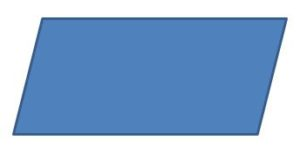The formula for the area of a parallelogram is identical to the formula for calculating the area of a rectangle because both are quadrilateral or four sided figures that have 2 sets of parallel and equal sides in terms of their lengths so, the formula for the area of a parallelogram, as shown in the picture below, is:

• Area = Base x HeightHere is an example of calculating the area of a parallelogram.

The parallelogram above has a base of 24 inches and a height of 11 inches. For the purpose of this exercise, we will call the top side of the parallelogram above as side 1, the lower side of this parallelogram as side 2, the left side of the parallelogram above as side 3 and the right side of this parallelogram as side 4, as shown above.

The calculation of the area of this parallelogram is done as follows:

• Area = Base x Height
• Area = 24 inches x 11 inches
• Area = 264 sq. inches

You can check this answer and also calculate the height or base of a parallelogram using this procedure, provided that you are given either the height or base of the parallelogram and its area:

• Area = Base x Height
• 264 sq. inches = 24 inches x Height
• Height = 264 divided by 24
• Height = 11 inches

As you can see above, you have checked your answer and found that 11 inches is the height of the parallelogram above. This means that the calculation of the area of the parallelogram above was correct and accurate. If you check your area calculation and end up with a base or height other than the one in the original area problem, you have either made an error in terms of calculating the area or an error in terms of calculating the height or base or both.

Here are some examples of calculating the area of a parallelogram.

Example 1:

What is the area of a parallelogram that has a base of 26 cm and a height of 6 cm?

• Area = Base x Height
• Area = 26 cm x 6 cm
• Area = 156 sq. cm
• Answer - The area of a parallelogram that has a base of 26 cm and a height of 6 cm is 156 sq. cm.

----------

Example 2:

What is the area of a parallelogram that has a base of 4 inches and a height of 987 inches?

• Area = Base x Height
• Area = 4 inches x 987 inches
• Area = 3,948 sq. inches
• Answer - The area of a parallelogram that has a base of 4 inches and a height of 987 inches is 3,948 sq. inches.

----------

Example 3:

What is the area of a parallelogram that has a base of 2.5 km and a height of 6 km?

• Area = Base x Height
• Area = 2.5 km x 6 km
• Area = 15 sq. km
• Answer - The area of a parallelogram that has a base of 2.5 km and a height of 6 km is 15 sq. km.

-----------

Example 4:

What is the area of a parallelogram that has a base of 13 cm and a height of 8 inches?

This example is different from the 3 above because the units of measurement (cm and inches) are not the same. For this example, you will have to convert the cm unit of measurement to the inches unit of measurement OR convert the inches unit of measurement to the cm unit of measurement.

For this example, we will convert the cm unit of measurement to the inches unit of measurement. There 0.39370079 cm in one inch. For the purpose of this practice example, 0.39370079 cm will be rounded off to 0.3937.

• 0.3937 cm : 1 inch = 13 cm : x inches
• 0.3937 x = 13
• x = 13 ÷ 0.3937
• x = 33.02 inches

Now that all the lengths in term of the height and the base are in the same unit of measurement (inches), you can now calculate the area of a parallelogram that has a base of 33.02 inches and a height of 8 inches.

• Area = Base x Height
• Area = 33.02 inches x 8 inches
• Area = 264.16 sq. inches
• Answer - The area of a parallelogram that has a base of 13 cm, which was converted to 33.02 inches, and a height of 8 inches is 264.16 sq. inches.

#### Performing Geometric Calculations with Parallelograms: Calculating the Base of Parallelogram Knowing the Area of a Parallelogram

In the same manner that you calculated the radius of a circle and the diameter of circles when the area of the circle is given to you in a problem, you can also calculate the length of the base of a parallelogram knowing the area of the parallelogram and the height of the parallelogram.

As you should recall, the rule to calculate the area of the parallelogram is:

• Area= Base x Height

Based on your knowledge of basic algebra, you would be able to calculate the length of the base of a parallelogram, as shown below for a parallelogram that has an area of 55 sq. inches and its height as 41 inches.

• Area= Base x Height
• 55 sq. inches = X x 41 inches
• x = 55 ÷ 41
• x = 1.3414 inches rounded off to the nearest tenth to 1.3 inches
• Answer - The length of the base of a parallelogram that has a height of 41 inches and an area of 55 sq. inches is 1.3 inches.

Here are some more example of calculating the length of the base of a parallelogram knowing the area of the parallelogram and the height of the parallelogram.

Example 1:

What is the length of the base of a parallelogram that has an area of 11 sq. km and a height as 3 km?

• Base = Area ÷ Height
• Base = 11 sq. km ÷ 3 km
• Base = 3.66 km
• Answer - The length of the base of a parallelogram that has a height of 3 km and an area of 11 sq. km is 3.66 km.

-----------

Example 2:

What is the length of the base of a parallelogram that has an area of 36 sq. feet and a height as 6 feet?

• Base = Area ÷ Height
• Base = 36 sq. feet ÷ 6 feet
• Base = 6 feet
• Answer - The length of the base of a parallelogram that has a height of 6 feet and an area of is 6 feet.

-----------

Example 3:

What is the length of the base of a parallelogram that has an area of 90 sq. inches and a height as 43 inches?

• Base = Area ÷ Height
• Base = 90 sq. inches ÷ 43 inches
• Base = 2.0930 or 2.1 inches rounded off the nearest tenth
• Answer - The length of the base of a parallelogram that has a height of 43 inches and an area of 90 sq. inches is 2.1 inches.

-----------

Example 4:

What is the length of the base of a parallelogram that has an area of 49 sq. inches and a height as 80 cm?

This example is different from the 3 above because the units of measurement (cm and inches) are not the same. For this example, you will have to convert the cm unit of measurement to the inches unit of measurement OR convert the inches unit of measurement to the cm unit of measurement.

For this example, we will convert the cm unit of measurement to the inches unit of measurement. There 0.39370079 cm in one inch. For the purpose of this practice example, 0.39370079 cm will be rounded off to 0.3937.

• 0.3937 cm : 1 inch = 80 cm : x inches
• x = 80 x 0.3937
• x = 31.496 inches
• x = 31.496 inches which rounded off to the nearest inch is 31 inches

Now that all the lengths in term of the height and the base are in the same unit of measurement (inches), you can now calculate the base of a parallelogram that has an area of 49 sq. inches and a height of 80 cm (31 inches).

• Base = Area ÷ Height
• x = 49 sq. inches ÷ 31 inches
• x = 1.5806
• Base = 1.5806 inches rounded off to the nearest tenth to 1.6 inches
• Answer - The length of the base of a parallelogram that has a height of 80 cm and an area of 49 sq. inches is 1.6 inches.

#### Performing Geometric Calculations with Parallelograms: Calculating the Height of Parallelogram Knowing the Area of a Parallelogram

In the same manner that you calculated the base of a parallelogram above, you can also calculate the length of the height of a parallelogram knowing the area of the parallelogram and the base of the parallelogram.

As you should recall, the rule to calculate the area of the parallelogram is:

• Area= Base x Height

Based on your knowledge of basic algebra, you would be able to calculate the length of the height of a parallelogram, as shown below for a parallelogram that has an area of 55 sq. inches and its base as 41 inches.

• Area= Base x Height
• 55 sq. inches = 41 inches x x inches
• x = 55 ÷ 41
• x = 1.3414 inches rounded off to the nearest tenth to 1.3 inches
• Answer - The length of the height of the parallelogram that has a base of 41 inches and an area of 55 sq. inches is 1.3 inches.

Here are some more examples of calculating the length of the base of a parallelogram knowing the area of the parallelogram and the height of the parallelogram.

Example 1:

What is the length of the base of a parallelogram that has an area of 33 sq. cm and a height as 12 cm?

• Area= Base x Height or
• Base = Area ÷ Height
• Base = 33 sq. cm ÷ 12 cm
• Base = 2.75 cm
• Answer - The length of the base of a parallelogram that has a height of 12 cm of and an area of 33 sq. cm is 2.75 cm.

-----------

Example 2:

What is the length of the base of a parallelogram that has an area of 2.8 sq. km and a height as 1 km?

• Area= Base x Height or
• Base = Area ÷ Height
• Base = 2.8 sq. km ÷ 1 km
• Base = 2.8 km
• Answer - The length of the base of a parallelogram that has a height of 1 km and an area of 2.8 sq. km is 2.8 km.

#### Performing Geometric Calculations with Parallelograms: Calculating Three Angles of Parallelogram Knowing the Angle of One of the Parallelograms' Angles

You are able to calculate and determine three of the four angles of a parallelogram when you know the measurement of only one of the four angles of a parallelogram,

This ability is based on some important facts relating to parallelograms and their characteristics. These facts are:

• A parallelogram has four sides.
• A parallelogram has four angles.
• The opposite angles of a parallelogram are equal.
• The adjacent angles of a parallelogram are complementary.
• The total of all the four angles of a parallelogram is 180 degrees.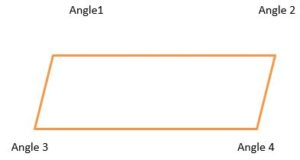Looking at the parallelogram above, angles 1 and 4 are opposite angles and angles 2 and 3 are opposite angles. Angles 1 and 4 are equal angles and angles 2 and 3 are equal angles.

Angles 1 and 2 are adjacent angles; angles 2 and 4 are adjacent angles; angles 3 and 4 are adjacent angles; and angles 3 and 1 are adjacent angles.

Because the adjacent angles of a parallelogram are complementary, because the opposite angles of a parallelogram are equal, and because the total of interior angles of a parallelogram total 180 degrees like a square and a rectangle, you can calculate the three angles of a parallelogram when you know only one of the four angles of a parallelogram.

Below are the procedure and the rationales for calculating the three angles of a parallelogram when you know only one of the four angles of a parallelogram:If angle 1 of the parallelogram above measures 30 degrees, what do angles 2, 3 and 4 measure?

Because angle 1 of the parallelogram above measures 30 degrees, angle 4 must also be 30 degrees because the opposite angles of a parallelogram are equal and angles 1 and 4 are opposite each other in the parallelogram above. Start here

Because angle 1 of the parallelogram above measures 30 degrees, angles 2 and 3 have to be 60 degrees because both angles 2 and 3 are adjacent to angles 1 and 4 and the adjacent angles of a parallelogram are complementary, that is, the adjacent angles of a parallelogram add up to a total of 90 degrees.

The angles of the above parallelogram are, therefore,:

Angle 1: 30 degrees (given information in the problem)

Angle 2: 60 degrees because adjacent angles of a parallelogram are complementary, that is, the adjacent angles of a parallelogram add up to a total of 90 degrees.

Angle 3: 60 degrees because adjacent angles of a parallelogram are complementary, that is, the adjacent angles of a parallelogram add up to a total of 90 degrees and because opposite angles 2 and 3 are equal. Angle 3, as you can see in the parallelogram above, is adjacent to angle 1 as well as angle 4.

Angle 4: 30 degrees because opposite angles 1 and 4, as opposite and equal angles on the parallelogram, are equal and also because angles 2 and 3 are complementary to angle 4, and as such, angle 4 plus angle 2 or 3, which are 60 degrees, is 90 degrees.

Here are some more examples:

Example 1:

If angle 2 of the parallelogram below measures 27 degrees, what do angles 1, 3 and 4 measure?Because angle 2 of the parallelogram above measures 27 degrees, angle 3 must also be 27 degrees because the opposite angles of a parallelogram are equal. Angles 2 and 3 are opposite and equal angles.

Because angle 2 of the parallelogram above measures 27 degrees, angles 1 and 4 have to be 63 degrees because both angles 1 and 4 are both adjacent to angle 2 and the adjacent angles of a parallelogram are complementary, that is, the adjacent angles of a parallelogram add up to a total of 90 degrees.

90 – 27 = 63

The angles of the above parallelogram are, therefore:

Angle 1: 63 degrees because angle 1 is adjacent to angle 2 and because adjacent angles of a parallelogram are complementary, that is, the adjacent angles of a parallelogram add up to a total of 90 degrees.

Angle 2: 27 degrees (given information in the problem)

Angle 3: 27 degrees because angle 3 is adjacent to angle 1 and because adjacent angles of a parallelogram are complementary, that is, the adjacent angles of a parallelogram add up to a total of 90 degrees and also because opposite angles 2 and 3 are equal.

Angle 4: 63 degrees because opposite angles 1 and 4 are opposite and equal angles and also because angles 2 and 3 are complementary to angle 4, and as such, angle 4 plus angle 2 or 3, which are 27 degrees, is 90 degrees.

-----------

Example 2:

If angle 1 of the parallelogram below measures 16 degrees, what do angles 2, 3 and 4 measure?Because angle 1 of the parallelogram above measures 16 degrees, angle 4 must also be 16 degrees because the opposite angles of a parallelogram are equal. Angles 1 and 4 are opposite and equal angles.

Because angle 1 of the parallelogram above measures 16 degrees, angles 2 and 3 have to be 74 degrees because both angles 1 and 4 are both adjacent to angle 1 and the adjacent angles of a parallelogram are complementary, that is, the adjacent angles of a parallelogram add up to a total of 90 degrees.

90 – 16 = 74

The angles of the above parallelogram are, therefore:

Angle 1: 16 degrees (given information in the problem)

Angle 2: 74 degrees because angle 2 is adjacent to angle 1 and because adjacent angles of a parallelogram are complementary, that is, the adjacent angles of a parallelogram add up to a total of 90 degrees.

Angle 3: 74 degrees because angle 3 is adjacent to angle 1 and because adjacent angles of a parallelogram are complementary, that is, the adjacent angles of a parallelogram add up to a total of 90 degrees and also because opposite angles 2 and 3 are equal.

Angle 4: 16 degrees because opposite angles 1 and 4 are opposite and equal angles and also because angles 2 and 3 are complementary to angle 4, and as such, angle 4 plus angle 2 or 3, which are 74 degrees, is 90 degrees.

-----------

Example 3:

If angle 3 of the parallelogram below measures 86 degrees, what do angles 1, 2 and 4 measure?Because angle 3 of the parallelogram above measures 86 degrees, angle 2 must also be 86 degrees because the opposite angles of a parallelogram are equal. Angles 3 and 4 are opposite and equal angles.

Because angle 3 of the parallelogram above measures 86 degrees, angles 1 and 4 have to be 4 degrees because both angles 1 and 4 are both adjacent to angle 3 and the adjacent angles of a parallelogram are complementary, that is, the adjacent angles of a parallelogram add up to a total of 90 degrees.

90 – 86 = 4

The angles of the above parallelogram are, therefore:

Angle 1: 4 degrees because angle 1 is adjacent to angle 2 and because adjacent angles of a parallelogram are complementary, that is, the adjacent angles of a parallelogram add up to a total of 90 degrees and also because opposite angles 1 and 4 are equal.

Angle 2: 86 degrees because angle 2 is adjacent to angle 1 and because adjacent angles of a parallelogram are complementary, that is, the adjacent angles of a parallelogram add up to a total of 90 degrees.

Angle 3: 86 degrees (given information in the problem)

Angle 4: 4 degrees because opposite angles 1 and 4 are opposite and equal angles and also because angles 2 and 3 are complementary to angle 4, and as such, angle 4 plus angle 2 or 3, which are 86 degrees, is 90 degrees

------------

Example 4:

If angle 4 of the parallelogram below measures 33 degrees, what do angles 1, 2 and 3 measure?Because angle 4 of the parallelogram above measures 33 degrees, angle 1 must also be 33 degrees because the opposite angles of a parallelogram are equal. Angles 1 and 4 are opposite and equal angles.

Because angle 1 of the parallelogram above measures 33 degrees, angles 2 and 3 have to be 57 degrees because both angles 2 and 3 are both adjacent to angle 1 and the adjacent angles of a parallelogram are complementary, that is, the adjacent angles of a parallelogram add up to a total of 90 degrees.

90 – 33 = 57

The angles of the above parallelogram are, therefore:

Angle 1: 33 degrees because angle 1 is adjacent to angle 2 and because adjacent angles of a parallelogram are complementary, that is, the adjacent angles of a parallelogram add up to a total of 90 degrees and also because opposite angles 1 and 4 are equal.

Angle 2: 57 degrees because angle 2 is adjacent to angle 1 and because adjacent angles of a parallelogram are complementary, that is, the adjacent angles of a parallelogram add up to a total of 90 degrees.

Angle 3: 57 degrees because opposite angles 2 and 3 are opposite and equal angles and also because angles 1 and 4 are complementary to angle 3, and as such, angle 3 plus angle 1 or 4, which are 33 degrees, is 90 degrees

Angle 4: 33 degrees (given information in the problem)

-----------

Example 5:

If angle 1 of the parallelogram below measures 7 degrees, what do angles 2, 3 and 4 measure?Because angle 1 of the parallelogram below measures 7 degrees, angle 4 must also be 7 degrees because the opposite angles of a parallelogram are equal. Angles 1 and 4 are opposite and equal angles.

Because angle 1 of the parallelogram above measures 7 degrees, angles 2 and 3 have to be 83 degrees because both angles 2 and 3 are both adjacent to angle 1 and the adjacent angles of a parallelogram are complementary, that is, the adjacent angles of a parallelogram add up to a total of 90 degrees.

90 – 7 = 83

The angles of the above parallelogram are, therefore:

Angle 1: 7 degrees (given information in the problem)

33 degrees because angle 1 is adjacent to angle 2 and because adjacent angles of a parallelogram are complementary, that is, the adjacent angles of a parallelogram add up to a total of 90 degrees and also because opposite angles 1 and 4 are equal.

Angle 2: 83 degrees because angle 2 is adjacent to angle 1 and because adjacent angles of a parallelogram are complementary, that is, the adjacent angles of a parallelogram add up to a total of 90 degrees.

Angle 3: 83 degrees because opposite angles 2 and 3 are opposite and equal angles and also because angles 1 and 4 are complementary to angle 3, and as such, angle 3 plus angle 1 or 4, which are 7 degrees, is 90 degrees

Angle 4: 7 degrees because opposite angles 4 and 1 are opposite and equal angles and also because angles 2 and 3 are complementary to angle 4, and as such, angle 4 plus angle 2 or 3, which are 83 degrees, is 90 degrees

### Trapezoid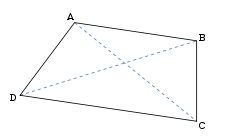A trapezoid is a quadrilateral with four sides and four angles like squares, parallelograms and rectangles, however, trapezoids, unlike squares, parallelograms and rectangles, trapezoids are characterized with at least one pair of opposite parallel sides. As you know, squares, parallelograms and rectangles have two pairs of opposite parallel sides. At times, a special type of a trapezoid has two pairs of opposite parallel lines but this does not occur often.

The opposite parallel sides of a trapezoid are called the bases of the trapezoid and the other sides that are not parallel are referred to as the legs or lateral sides.

A trapezoid has two acute angles and two obtuse angles, unless it is a special type of trapezoid called a right trapezoid, which would then have two right angles of 90 digress, one acute angle and one obtuse angle.

There are several types of trapezoids. They are:

#### Acute Trapezoid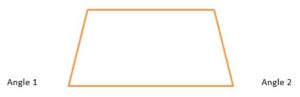An acute trapezoid has two angles along its longer base that are two acute angles of less than 90 degrees, as shown below as angles 1 and 2. You should also be able to see in the trapezoid below that the top and the bottom of the trapezoid are parallel bases and the two lateral sides are not parallel.

#### Obtuse Trapezoid

An obtuse trapezoid has two angles that are greater than 90 degrees, as shown in the upper right hand corner of the picture below.

#### Right Trapezoid

A trapezoid that has two right angles adjacent or next to each other. A right trapezoid is also referred to as a right angled trapezoid. A right trapezoid is shown in the picture immediately above.

#### Isosceles Trapezoid

A special type of an acute trapezoid that has two of its opposite bases parallel and the two legs or sides of the trapezoid equal in terms of their length. See the picture below.

##### Performing Geometric Calculations with Trapezoids: Calculating the Perimeter of a Trapezoid

The formula for the perimeter of a trapezoid is identical to the formula for calculating the perimeter of a rectangle and a parallelogram because they are all quadrilaterals or four sided figures that have at least one set of parallel sides or bases.

The formula for the perimeter of a trapezoid is:

Perimeter of a trapezoid = Base 1 + Base 2 + Lateral Side 1 + Lateral Side 2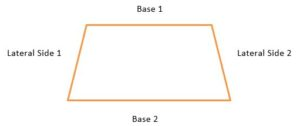Here is an example of calculating the perimeter of a trapezoid.

The measurements of the above trapezoid are:

• Base 1: 15 cm
• Base 2: 30 cm
• Lateral Side 1: 9 cm
• Lateral Side 2: 10 cm
• Perimeter of a trapezoid = Base 1 + Base 2 + Lateral Side 1 + Lateral Side 2
• Perimeter of the trapezoid = 15 cm + 30 cm + 9 cm + 10 cm
• Perimeter of the trapezoid = 64 cm

Here are some more practice examples relating to the perimeter of a trapezoid.

Example 1:

What is the perimeter of a trapezoid with the following measurements?

• Base 1: 4 inches
• Base 2: 11 inches
• Lateral Side 1: 6 inches
• Lateral Side 2: 9 inches
• Perimeter of a trapezoid = Base 1 + Base 2 + Lateral Side 1 + Lateral Side 2
• Perimeter of the trapezoid = 4 inches + 11 inches + 6 inches + 9 inches = 30 inches
• Answer - The perimeter of this trapezoid is 30 inches.

----------

Example 2:

What is the perimeter of a trapezoid with the following measurements?

• Base 1: 22 cm
• Base 2: 18 cm
• Lateral Side 1: 9 cm
• Lateral Side 2: 8 cm
• Perimeter of a trapezoid = Base 1 + Base 2 + Lateral Side 1 + Lateral Side 2
• Perimeter of the trapezoid = 22 cm + 18 cm + 9 cm + 8 cm = 57 cm
• Answer - The perimeter of this trapezoid is 57 cm.

-----------

Example 3:

What is the perimeter of a trapezoid with the following measurements?

Base 1: 4 km

Base 2: 6 km

Lateral Side 1: 3 km

Lateral Side 2: 3 km

Perimeter of a trapezoid = Base 1 + Base 2 + Lateral Side 1 + Lateral Side 2

Perimeter of the trapezoid = 4 km + 6 km + 3 km + 3 km = 16 km

Answer - The perimeter of this trapezoid is 16 km.

##### Performing Geometric Calculations with Trapezoids: Calculating the Area of a Trapezoid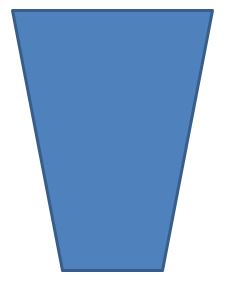The area of a trapezoid, as highlighted above in blue, is calculated with the following formula:

• Area of a Trapezoid = (Length of Base 1 + Length of Base 2) x h/2

For example, the calculation for the area of a trapezoid is done as below, when the measurements of the trapezoid are:

• Length of Base 1 = 22 cm
• Length of Base 2 = 18 cm
• Height = 15
• Area of a Trapezoid = (Length of Base 1 + Length of Base 2) x h/2
• Area of a Trapezoid = (22 cm + 18 cm) x 15/2
• Area of a Trapezoid = 40 cm x 7.5 = 300 sq. cm
• Answer - The area of the trapezoid that has the measurements above is 300 sq. cm

Now, here are some practice problems for calculating the area of a trapezoid.

Example 1:

Calculate the area of a trapezoid that has the following measurements:

• Length of Base 1 = 7 km
• Length of Base 2 = 3.5 km
• Height = 2 km
• Area of a Trapezoid = (Length of Base 1 + Length of Base 2) x h/2
• Area of a Trapezoid = (7 km + 3.5 km) x 2/2
• Area of a Trapezoid = 10. 5 km x 1 = 10. 5 sq. km
• Answer - The area of the trapezoid that has the measurements above is 10. 5 sq. km.

------------

Example 2:

Calculate the area of a trapezoid that has the following measurements:

• Length of Base 1 = 13.3 feet
• Length of Base 2 = 66.3 feet
• Height = 45 feet
• Area of a Trapezoid = (Length of Base 1 + Length of Base 2) x h/2
• Area of a Trapezoid = (13.3 feet + 66.3 feet) x 45/2
• Area of a Trapezoid = 79.6 feet x 22.5 feet = 1,791 sq. feet
• Answer - The area of the trapezoid that has the measurements above is 1,791 sq. feet.

----------

Example 3:

Calculate the area of a trapezoid that has the following measurements:

• Length of Base 1 = 45 cm
• Length of Base 2 = 21 inches
• Height = 50 cm

For this example, you have to convert inches into cm or cm into inches so that you have the same units of measurement. Since both the height and the length of base 2 are in cm, the easiest and quickest way to convert to identical units of measurement is to convert the 21 inches into cm, as follows:

• 1 inch : 2.54 cm = 21 inches : x cm
• x = 2.54 x 21
• x = 53.35 cm

Now, you can calculate the area of the trapezoid using the cm unit of measurement, as below.

• Area of a Trapezoid = (Length of Base 1 + Length of Base 2) x h/2
• Area of a Trapezoid = (45 cm + 53.35 cm) x 50/2
• Area of a Trapezoid = 98.35 cm x 25 cm= 2,458.75 sq. cm
• Answer - The area of the trapezoid that has the measurements above is 2,458.75 sq. cm.

## Lines & Angles

### Lines

Lines extend from one point to another to infinity. In other words, lines can be long or short, as shown in the picture below.

____________________________

___________

______________________________________________________________

### Angles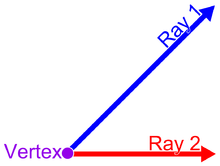An angle formed by two rays emanating from a vertex.

Simply stated, an angle is created when two straight lines share and have a common origin. The wo straight lines of an angle are referred to as rays and the point of common origin is called the vertex.

Lines that create angles are NOT parallel to each other. Parallel lines, unlike rays that form an angle, can go on to infinity without touching at a point of common origin or vertex. Below is an example of parallel lines that do not share a common vertex. As you can see, these parallel lines are quite unlike the rays above that form an angle.

____________________________

___________

Angles can be characterized and classified in several ways. Angles can be described as a:

• Straight angle
• Right angle
• Acute angle
• Obtuse angle
• Complementary angle
• Supplementary angle
• Explementary angle

#### Straight Angles

Straight angle are a flat line. Lines that are parallel, as shown above, have straight angles. A straight angle is 180 degrees.

#### Right AnglesA right angle is equal to 90 degrees.

Right angles are angles that are formed when the rays, or lines, that form the angle are perpendicular to each other. An example of right angles in our everyday life that are formed by rays or lines that are perpendicular to each other include a cross, the addition plus sign (+) and a graph that shows the x axis and the y axis at the point of origin as shown below. In the case of the graph, the rays are the x axis and the y axis and the vertex is the point of origin, as seen on the graph immediately below.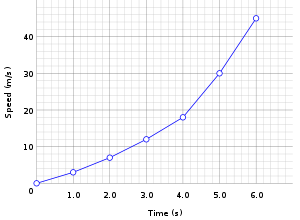The graph below has four quadrants rather than the one quadrant formed by the two rays above. The graph below has four right angles formed by the x and y axes. Each right angle is shown in the inner part of this graph at the point of origin where these axes form from a vertex.The lines, or rays, shown in the picture below show another variation of right angles.

##### Comparing and Contrasting Straight Angles and Right Angles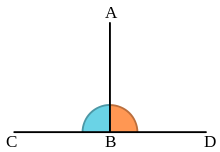A line segment (AB) drawn so that it forms right angles with a line (CD).

As you can see in the picture above, the orange and blue colored areas are right angles, each of which is 90 degrees. When these orange and blue colored right arrows are combined, they form a straight angle of 180 degrees along the horizontal line labeled C D.

#### Acute AnglesAn angle formed by two rays emanating from a vertex.

Acute angles, similar to right angles, are formed by two lines, or rays, at the common and shared vertex. However, acute angles are not similar to right angles in that a right angle is always exactly 90 degrees and an acute angle is NEVER 90 degrees and it is ALWAYS less than 90 degrees.

Acute angles, therefore, can range from 89.99999 to zero degrees and all other angles less than 90 degrees. They have a sharp, acute, point at the vertex.

#### Obtuse Angles

Obtuse angles, similar to right angles, are formed by two lines, or rays, at the common and shared vertex. However, acute angles are not similar to right angles in that a right angle is always exactly 90 degrees and an obtuse angle is NEVER 90 degrees and it is ALWAYS more than 90 degrees but always less than 180 degrees which is a flat line.

Obtuse angles, in contrast to acute and right angles are flattened in terms of their appearance and not sharp like an acute angle and they are not upright and perpendicular to a horizontal line like a right angle.

The angle labeled b in the picture below is an obtuse angle that is greater than 90 degrees; the angle labeled a in the picture below is an acute angle that is less than 90 degrees; and the angle labeled c in the picture below is a straight angle that is precisely 180 degrees.Acute (a), obtuse (b), and straight (c) angles. The acute and obtuse angles are also known as oblique angles.

Simply defined, an adjacent angle is an angle that shares a common vertex side by side. These adjacent angles do NOT have to be equal in terms of their width and number of degrees; they do NOT have to add up to 90 degrees; and they do NOT have to add up to 180 degrees.Angles A and B are adjacent.

Adjacent angles like the ones above, labeled angles A and B, are adjacent angles. There are three different types or subcategories of adjacent angles. They are complementary angles, supplementary angles, and explementary angles. These types of adjacent angles are discussed immediately below.

#### Complementary Angles

Complementary angles, which are a special type of adjacent angles, are angles that share a common vertex side by side with each other and ALSO combine to form a right angle of 90%. Complementary angles do NOT have to be equal in terms of their degrees, but the sum of their degrees must add up to 90 degrees.

For example, if you have 2 complementary angles and one is 40 degrees, the other must be 50 degrees because these two acute angles MUST add up to 90 degrees. If you are asked to identify the number of degrees that an angle has and you are told that its complementary angle is 30 degrees, you should know that this angle measures 60 degrees because:

x + 30 = 90 and

x = 90 – 30

x = 60 degrees

#### Supplementary AnglesA line segment (AB) drawn so that it forms right angles with a line (CD).

Supplementary angles, which are also a special type of adjacent angles, are angles that share a common vertex side by side with each other and they ALSO combine to form a straight angle of 180%. Supplementary angles do NOT have to be equal in terms of their degrees, but the sum of their degrees must add up to 180 degrees.

As you can see in the picture above, the orange colored angle and the blue colored angle are supplementary angles that share the vertex at point B on the line. These orange colored and blue colored angles happen to be each a right angle, but the fact that supplementary angles are right angles of 90 degrees is NOT necessary to make them supplementary angles. The fact that the two angles add up to 180 degrees makes then supplementary angles.The angles a and b are supplementary angles

For example, if you have 2 supplementary angles and one is 140 degrees, the other must be 40 degrees because these two angles MUST add up to 180 degrees. If you are asked to identify the number of degrees that an angle has and you are told that its supplementary angle is 110 degrees, you should know that this angle measures 70 degrees because:

x + 110 = and

x = 180 – 110

x = 70 degrees

#### Explementary Angles

Explementary angles, which are also a special type of adjacent angles, are angles that share a common vertex side by side with each other, similar to complementary and supplementary angles, but they combine to form an angle of 360 degrees and not the 90 degrees and 180 degrees that complementary and supplementary angles have, respectively.

Explementary angles do NOT have to be equal in terms of their degrees, but the sum of their degrees must add up to 360 degrees.

For example, if you have 2 explementary angles and one is 210 degrees, the other must be 150 degrees because these two angles MUST add up to 360 degrees. If you are asked to identify the number of degrees that an angle has and you are told that its explementary angle is 190 degrees, you should know that this angle measures 170 degrees because:

x + 190 = 360 and

x = 360 – 190

x = 170 degrees

RELATED TEAS MEASUREMENT & DATA CONTENT:Latest posts by Alene Burke, RN, MSN (see all)